# NCERT Exemplar Solutions for Class 12 Maths Chapter 6 Application of Derivatives

Subject-wise textbooks of the NCERT Exemplar for Class 12 are important for students to prepare for the board exam. Solving questions from the Exemplar will strengthen conceptual understanding among students. To help students understand the subject better, NCERT Exemplar Solutions, prepared by subject experts at BYJU’S, is the right resource. Also, these solutions are in accordance with the latest CBSE guidelines.

The sixth chapter of NCERT Exemplar Solutions for Class 12 Maths is the Application of Derivatives. In this chapter, students will learn how to solve problems of derivatives based on the rate of change of quantitative, increasing and decreasing functions, tangents and normals, approximation, maxima and minima, and maximum and minimum values of a function in a closed interval. Students who aspire to attain a strong grip over the concepts of this chapter can access the solutions PDF of NCERT Exemplar Solutions for Class 12 Maths Chapter 6 Application of Derivatives from the link below.

## Download the PDF of NCERT Exemplar Solutions for Class 12 Maths Chapter 6 Application of Derivatives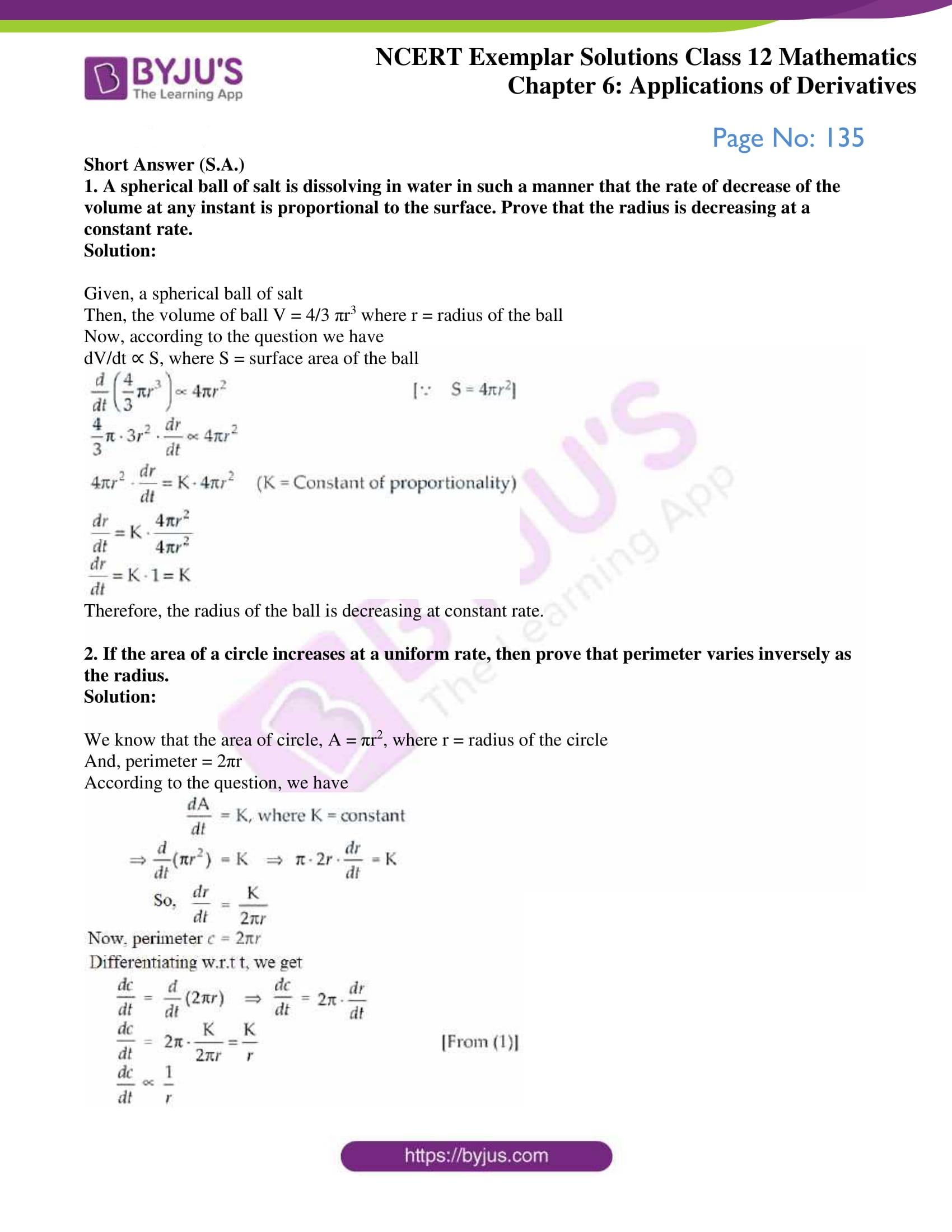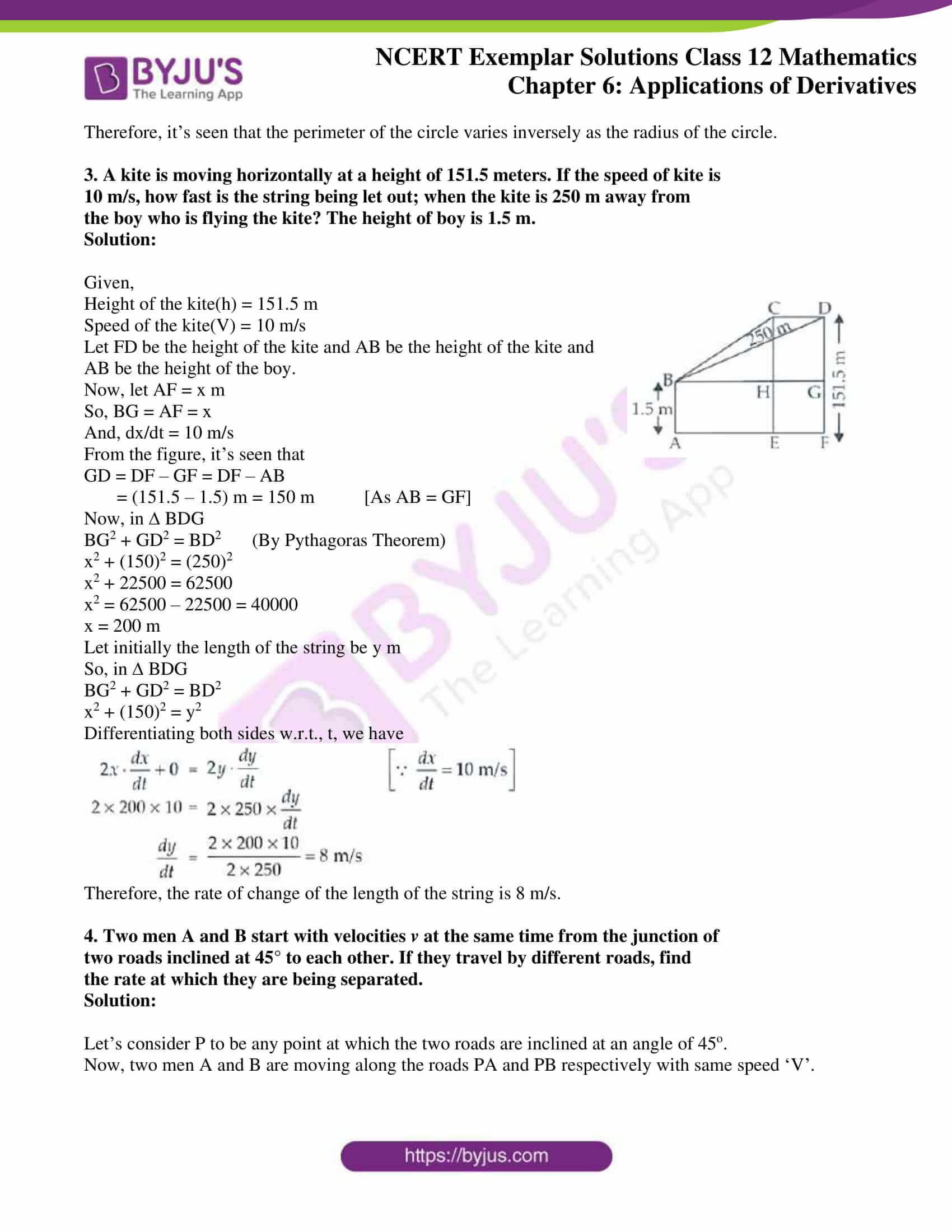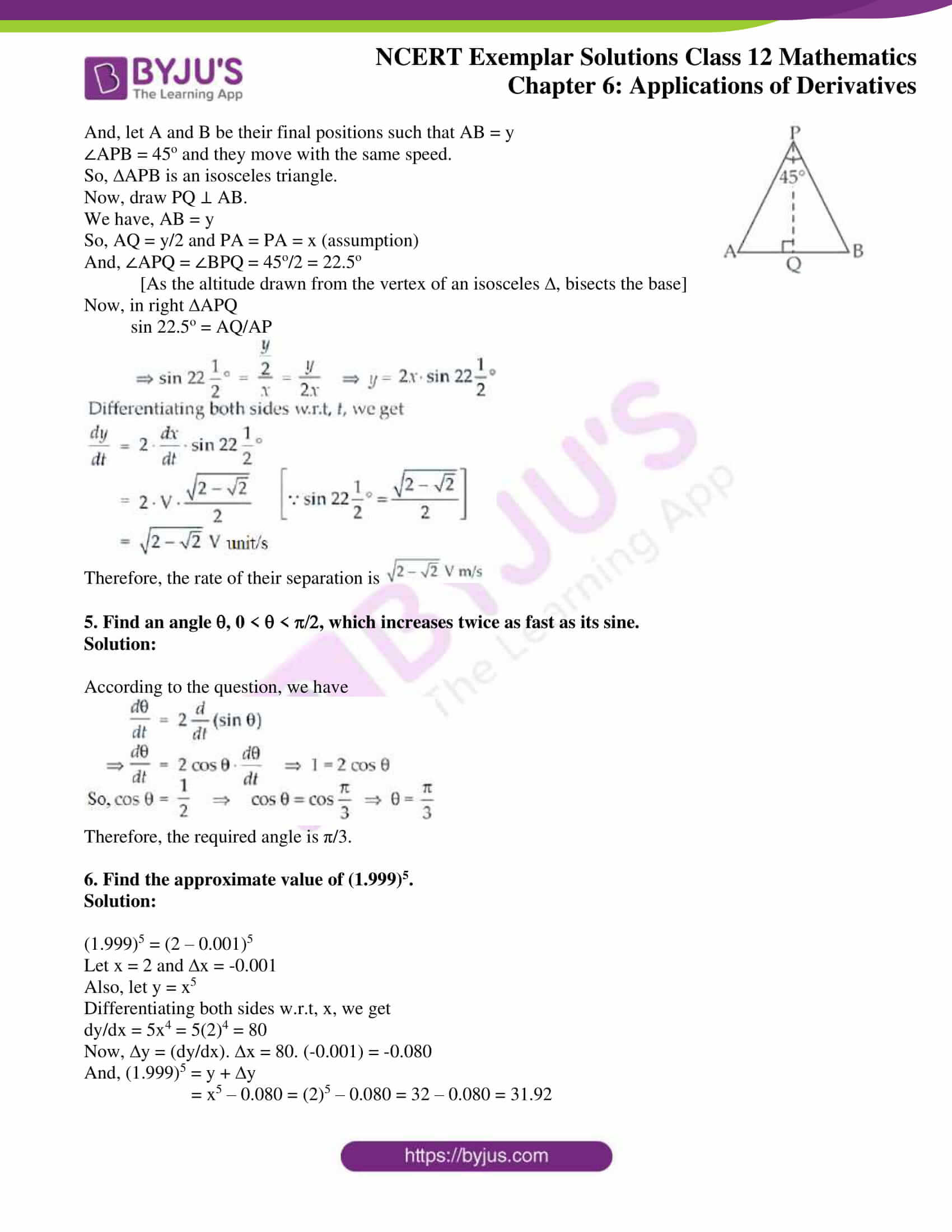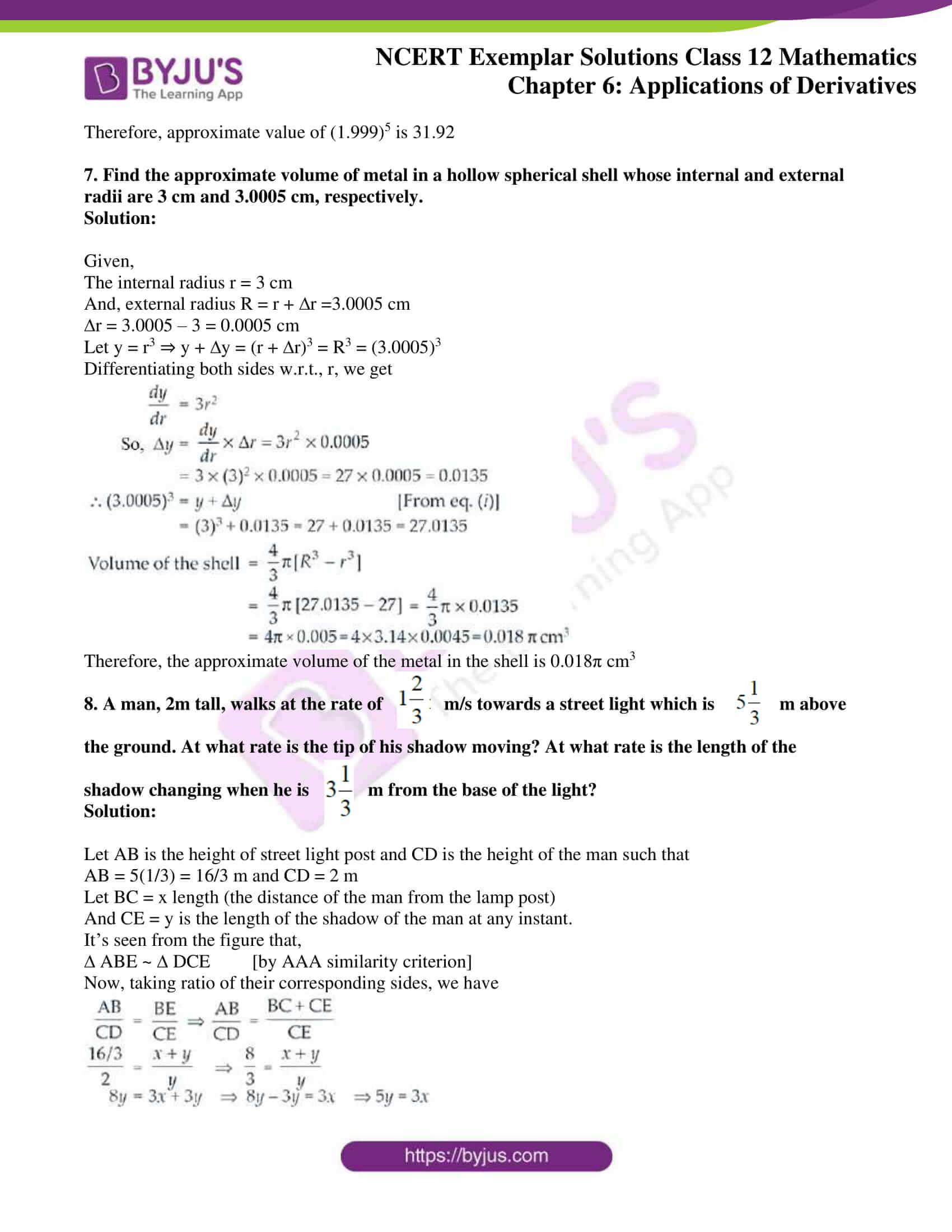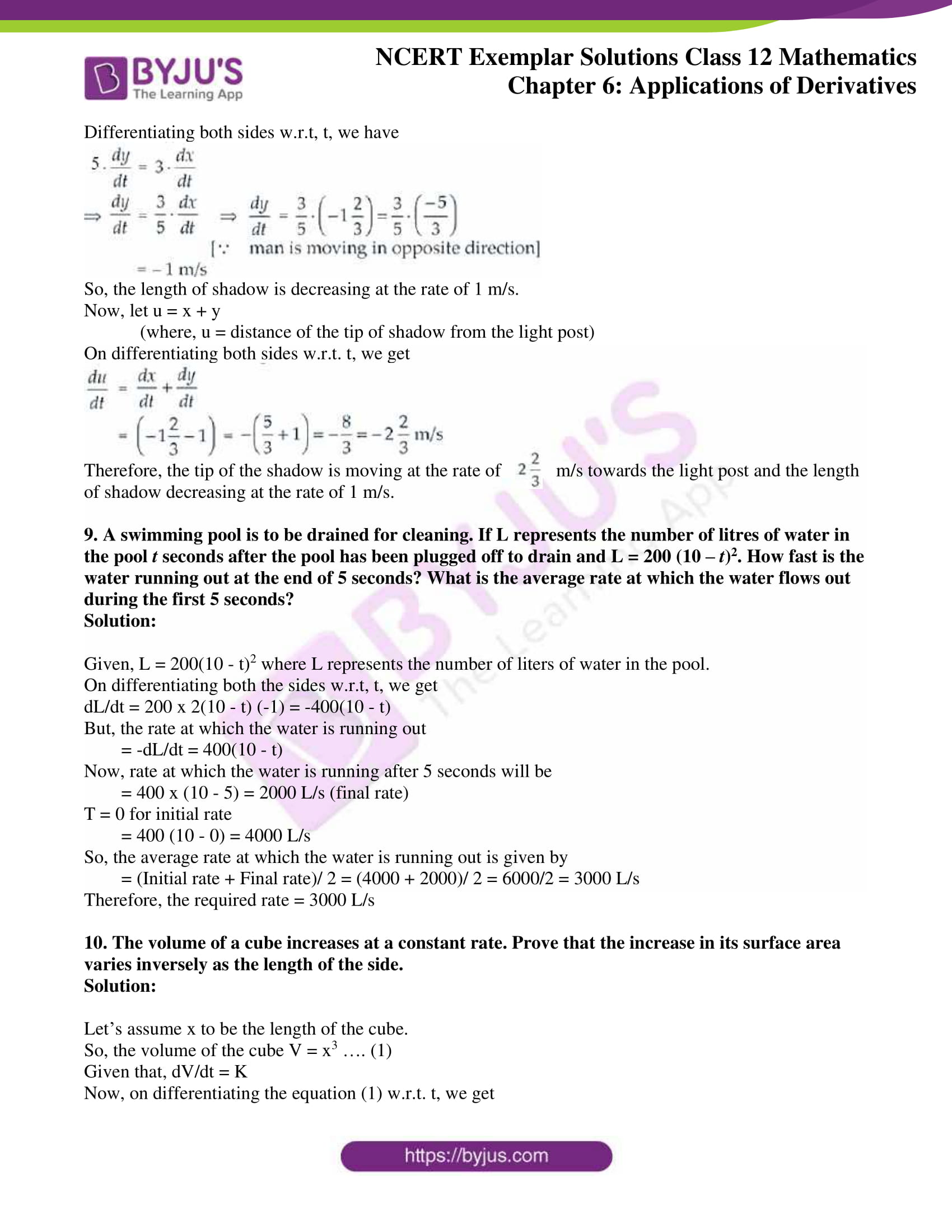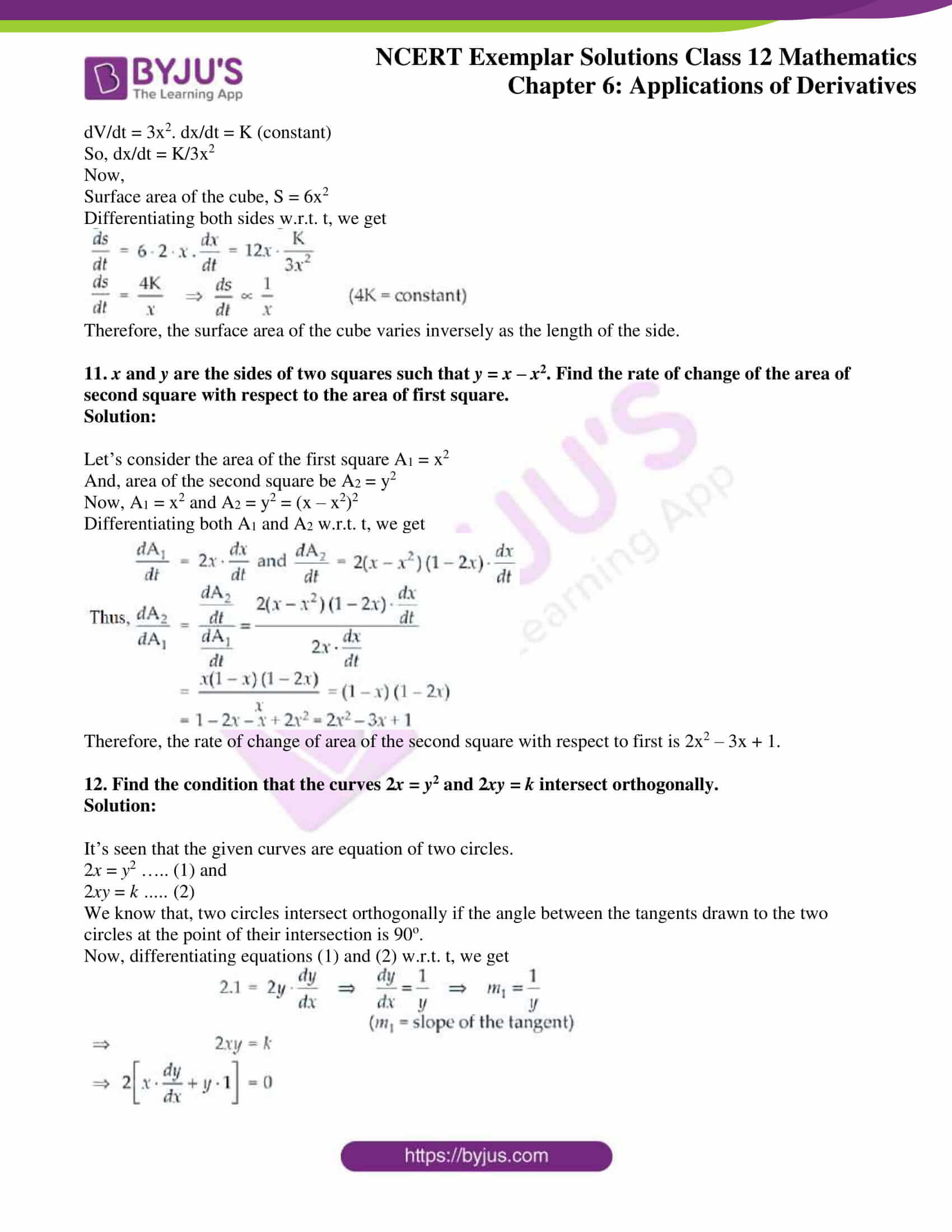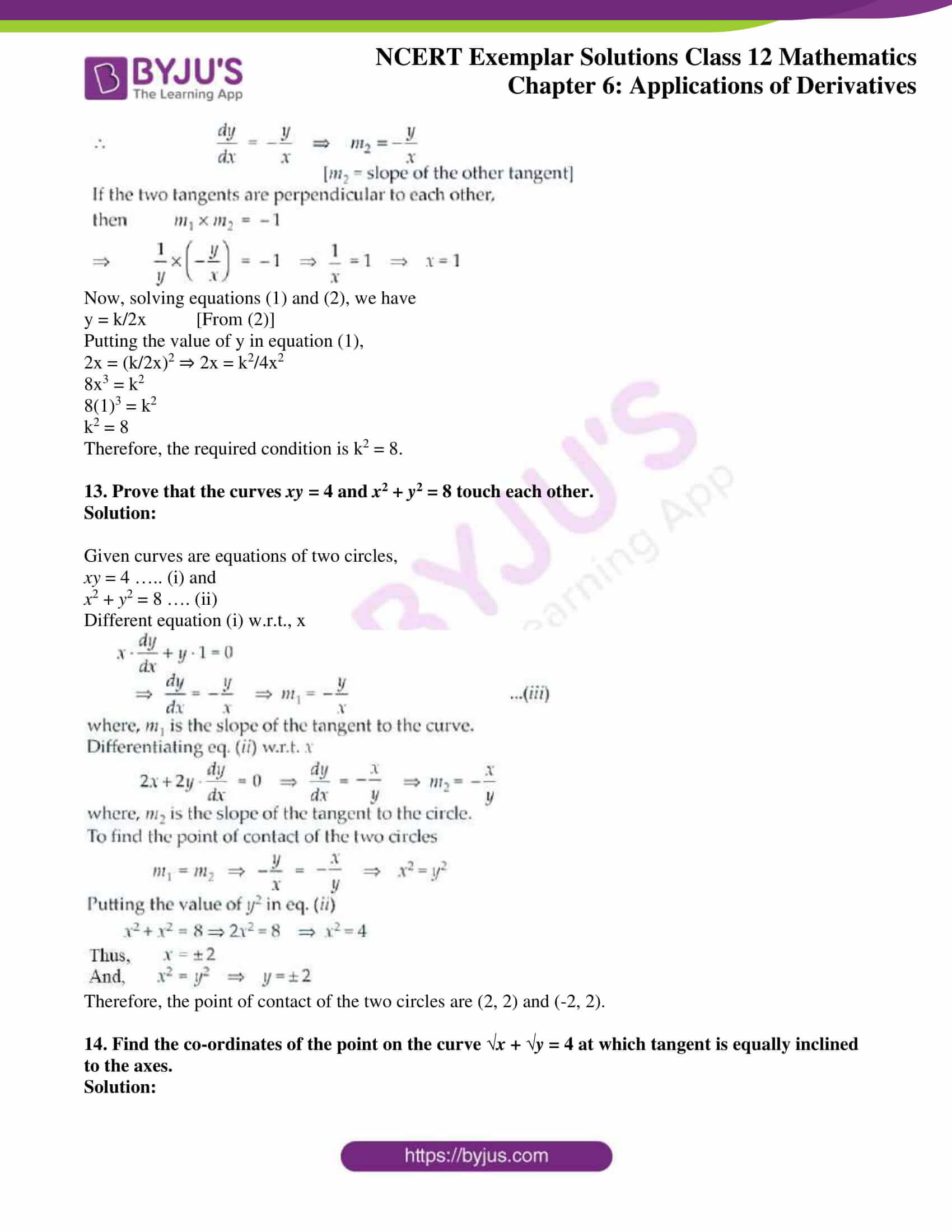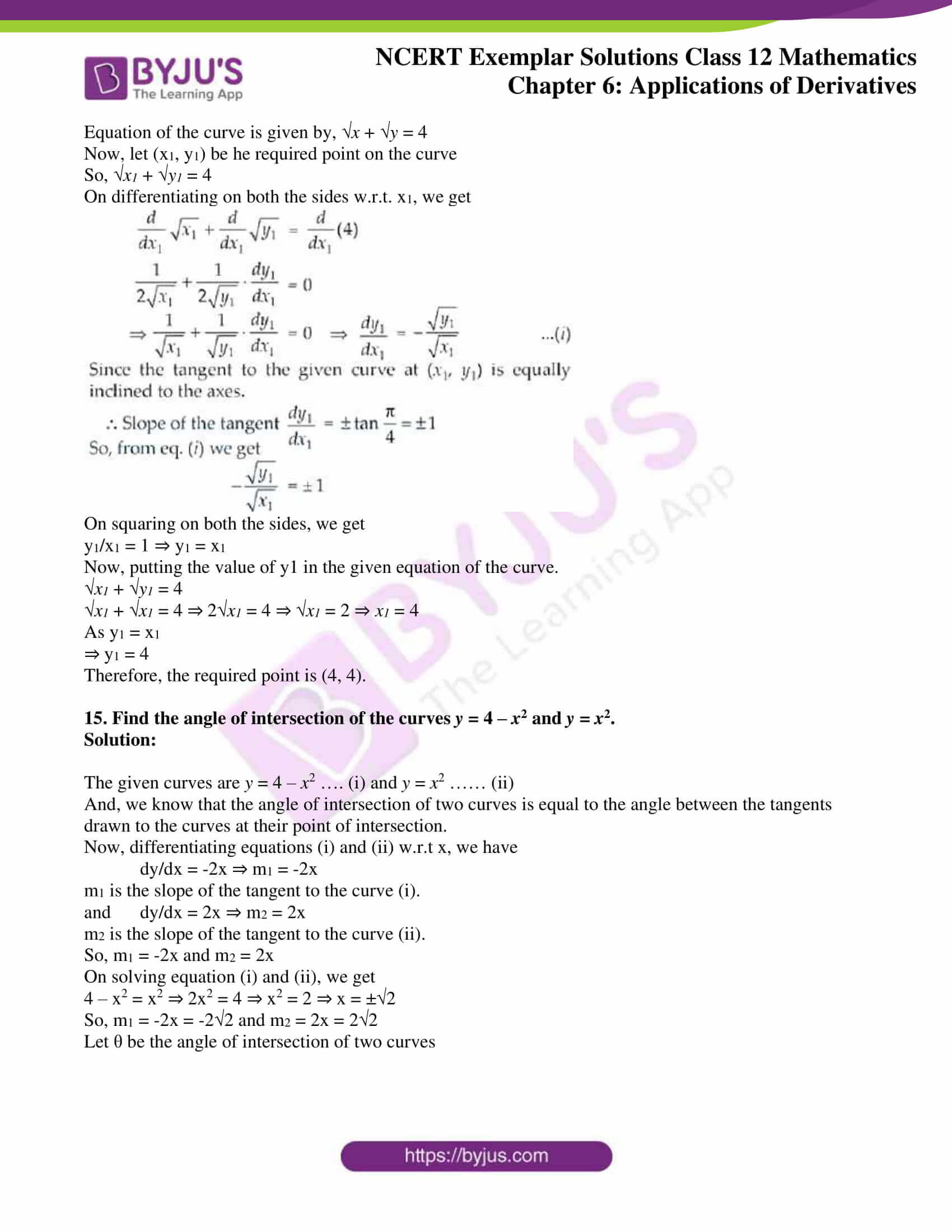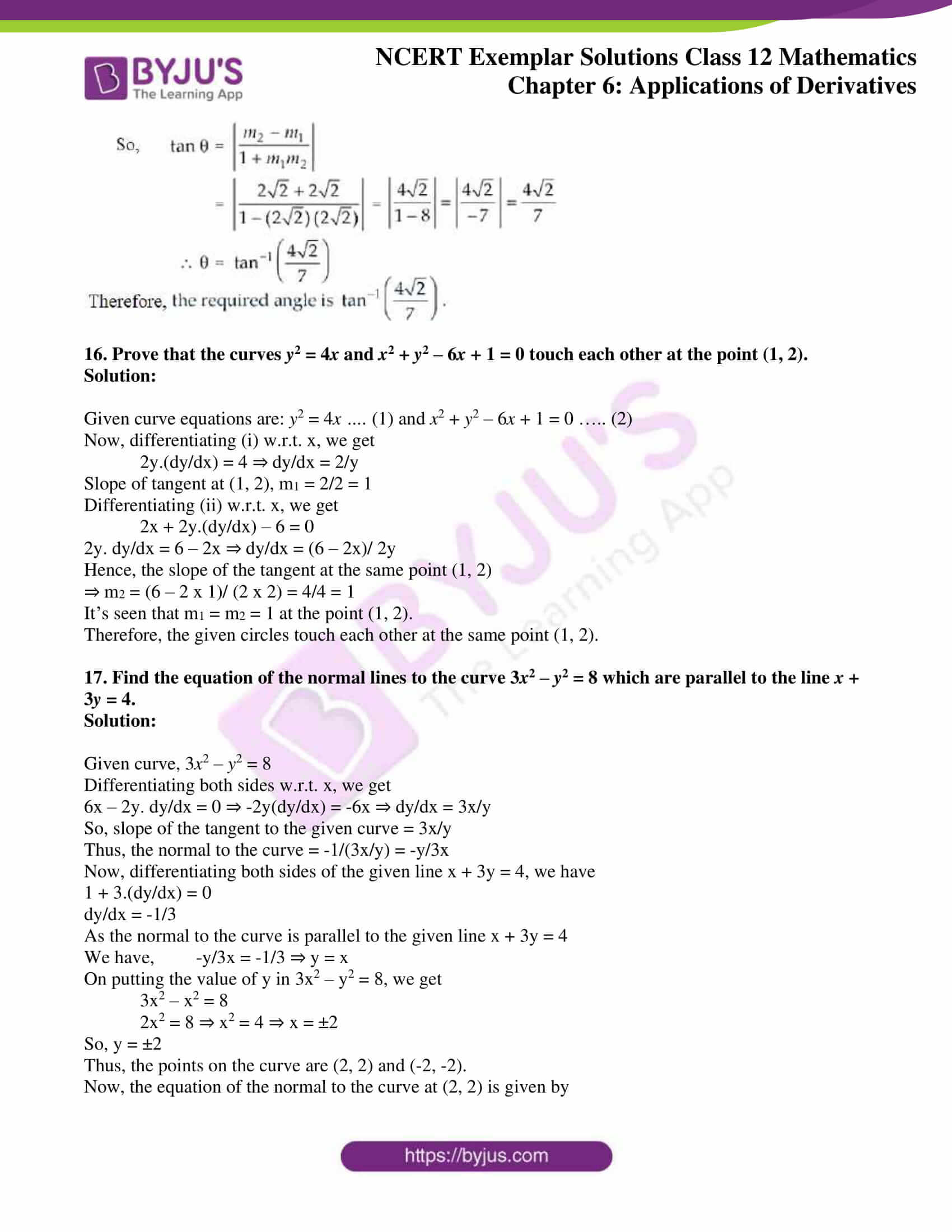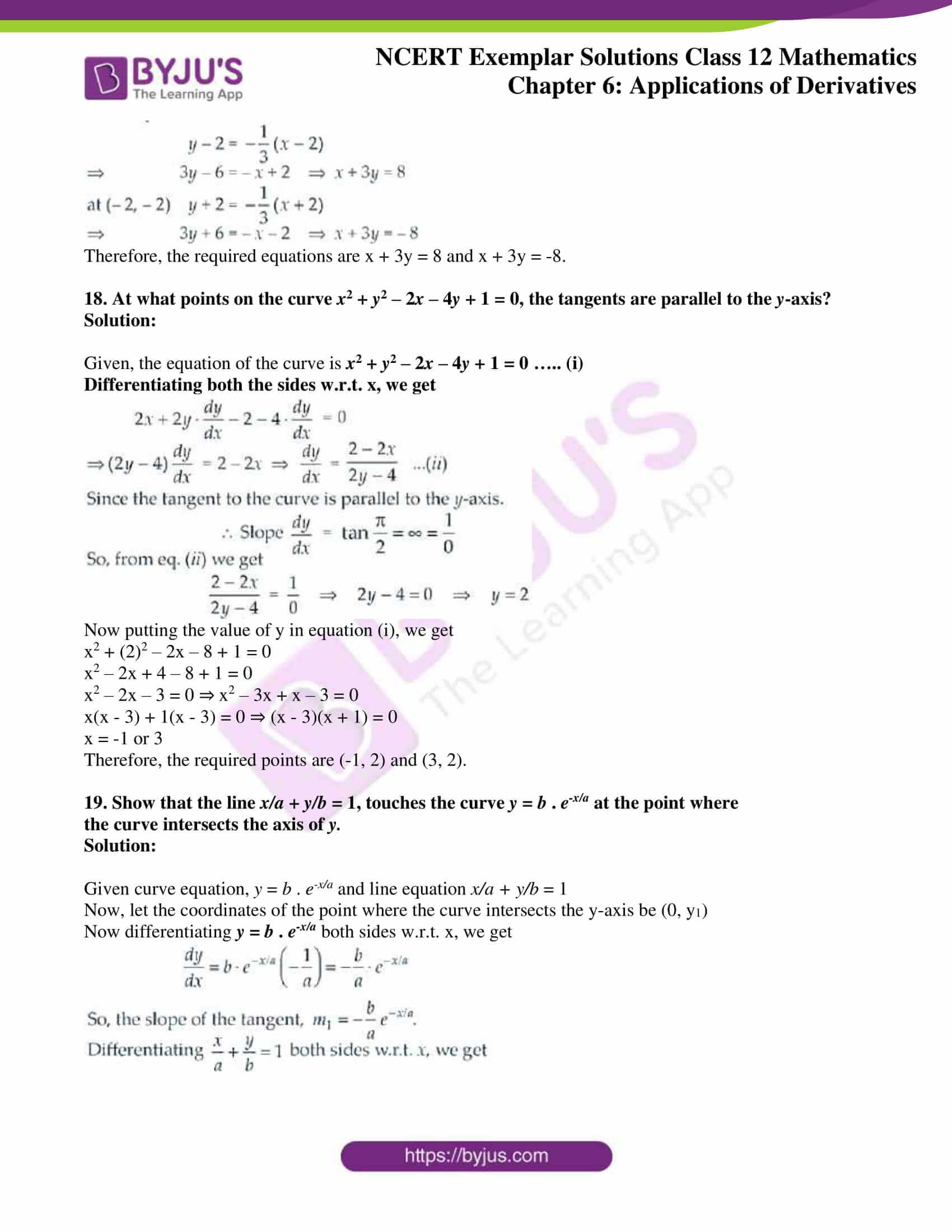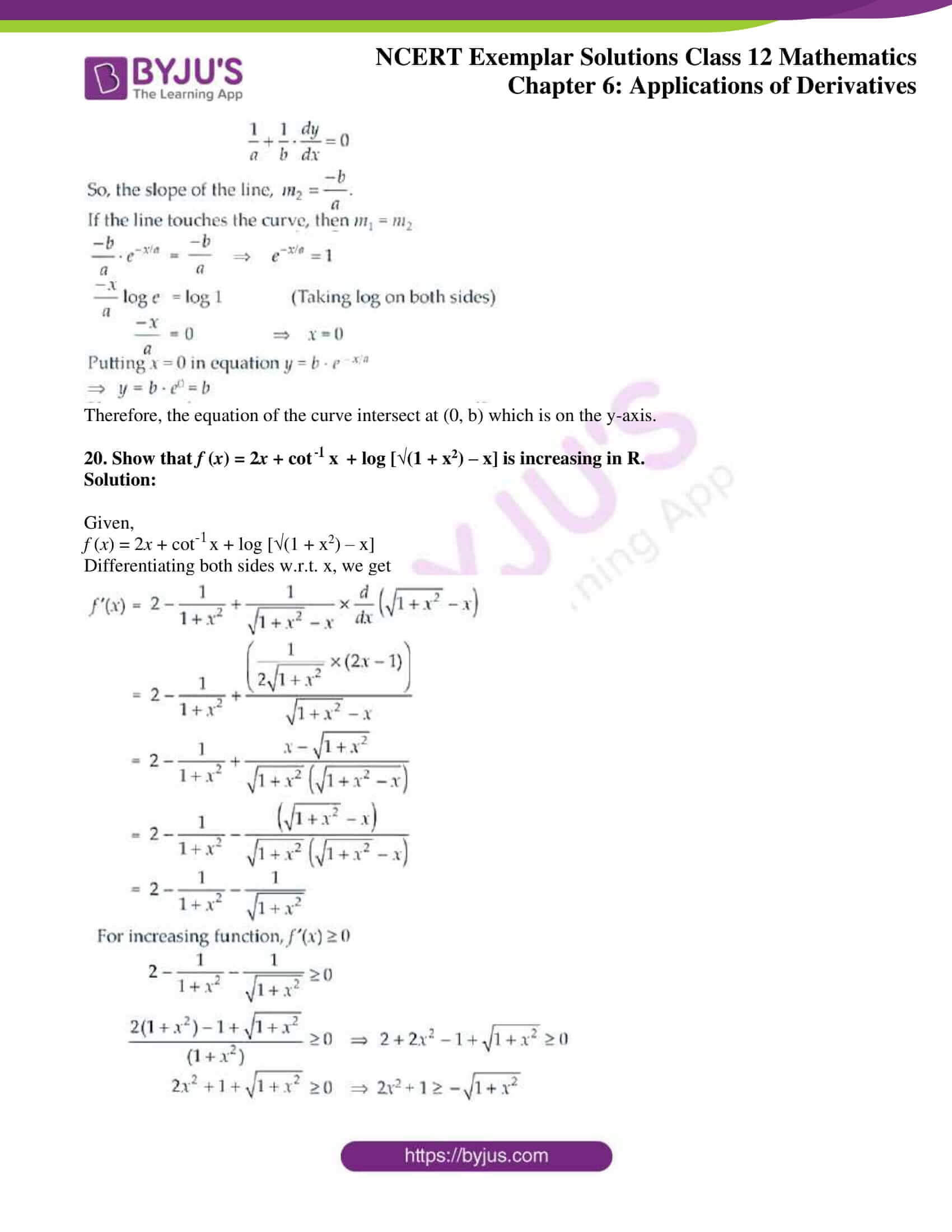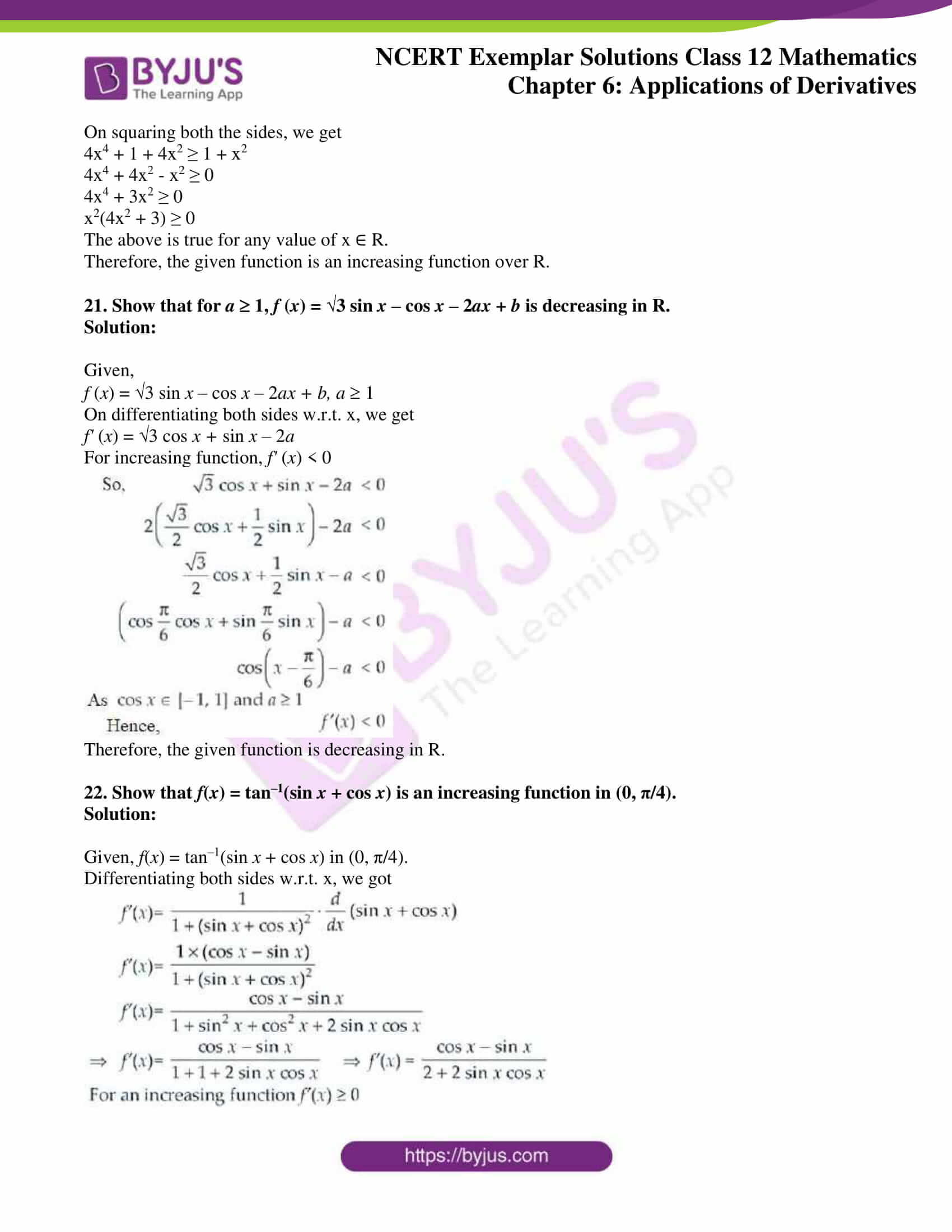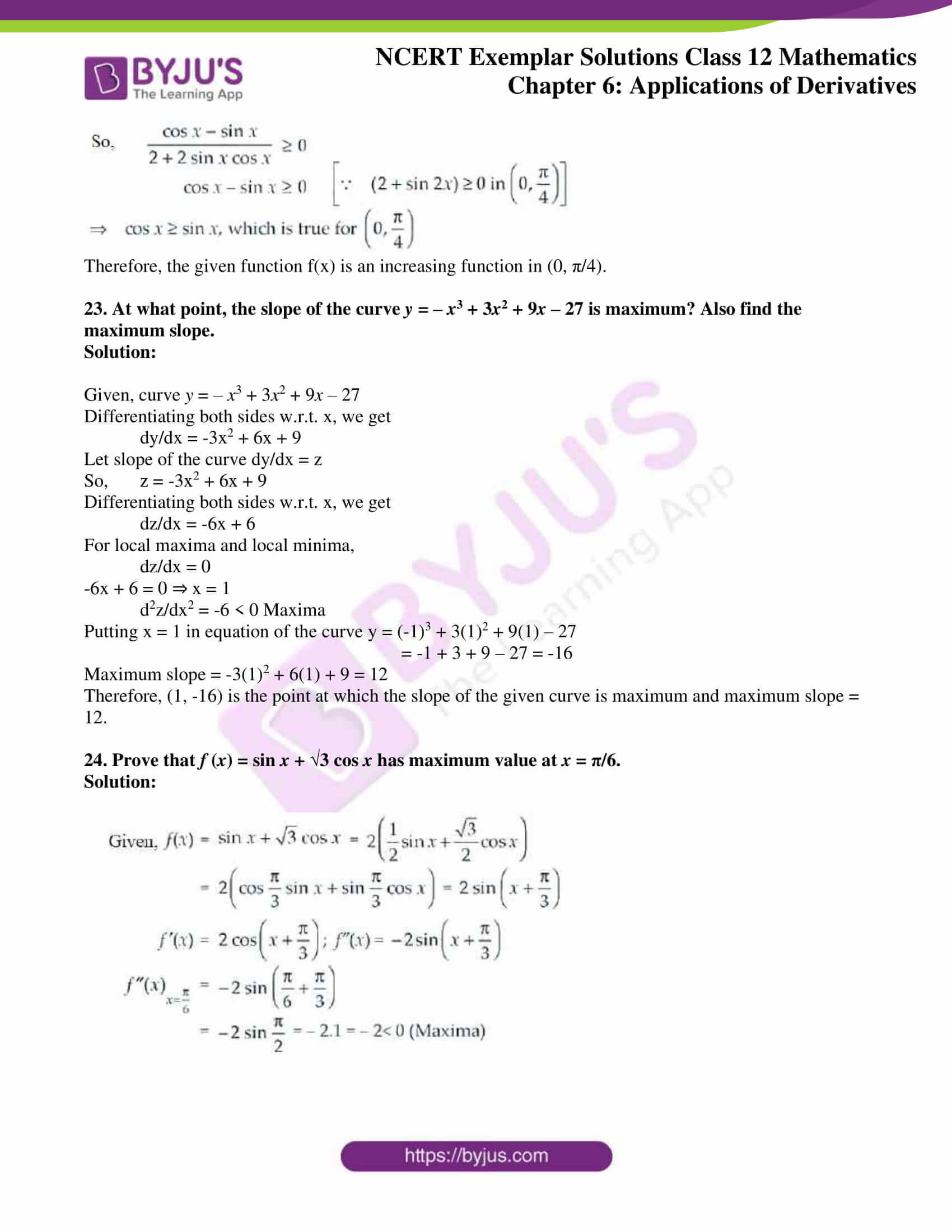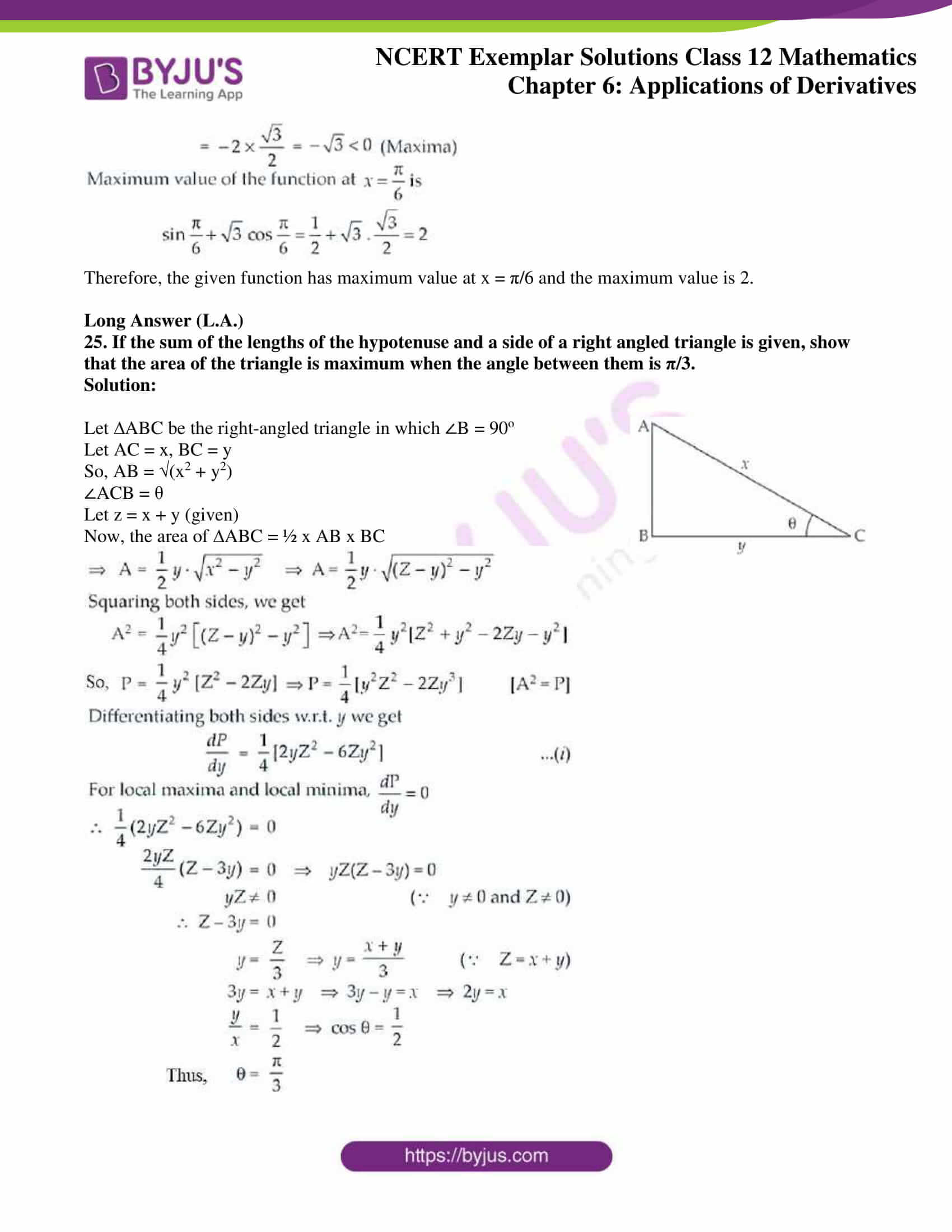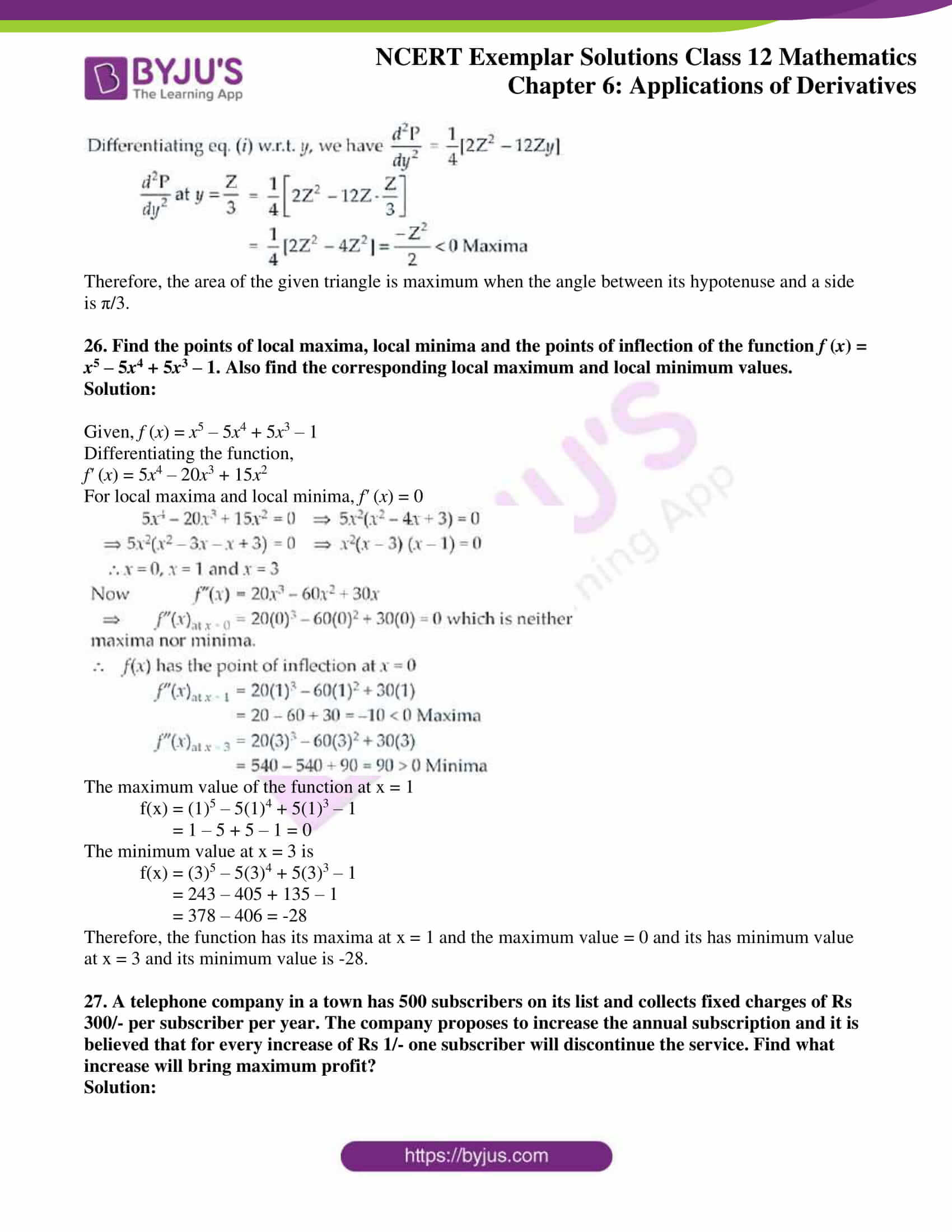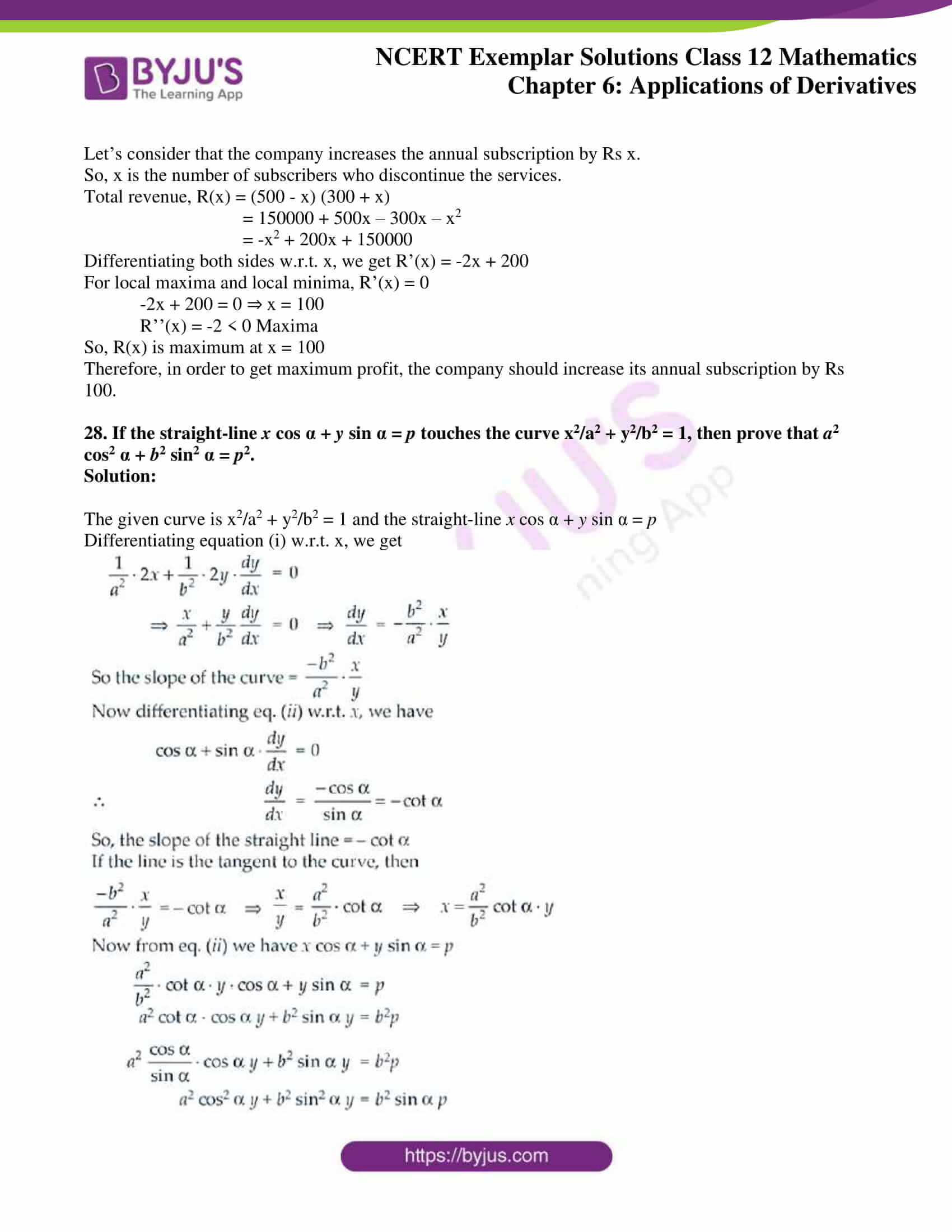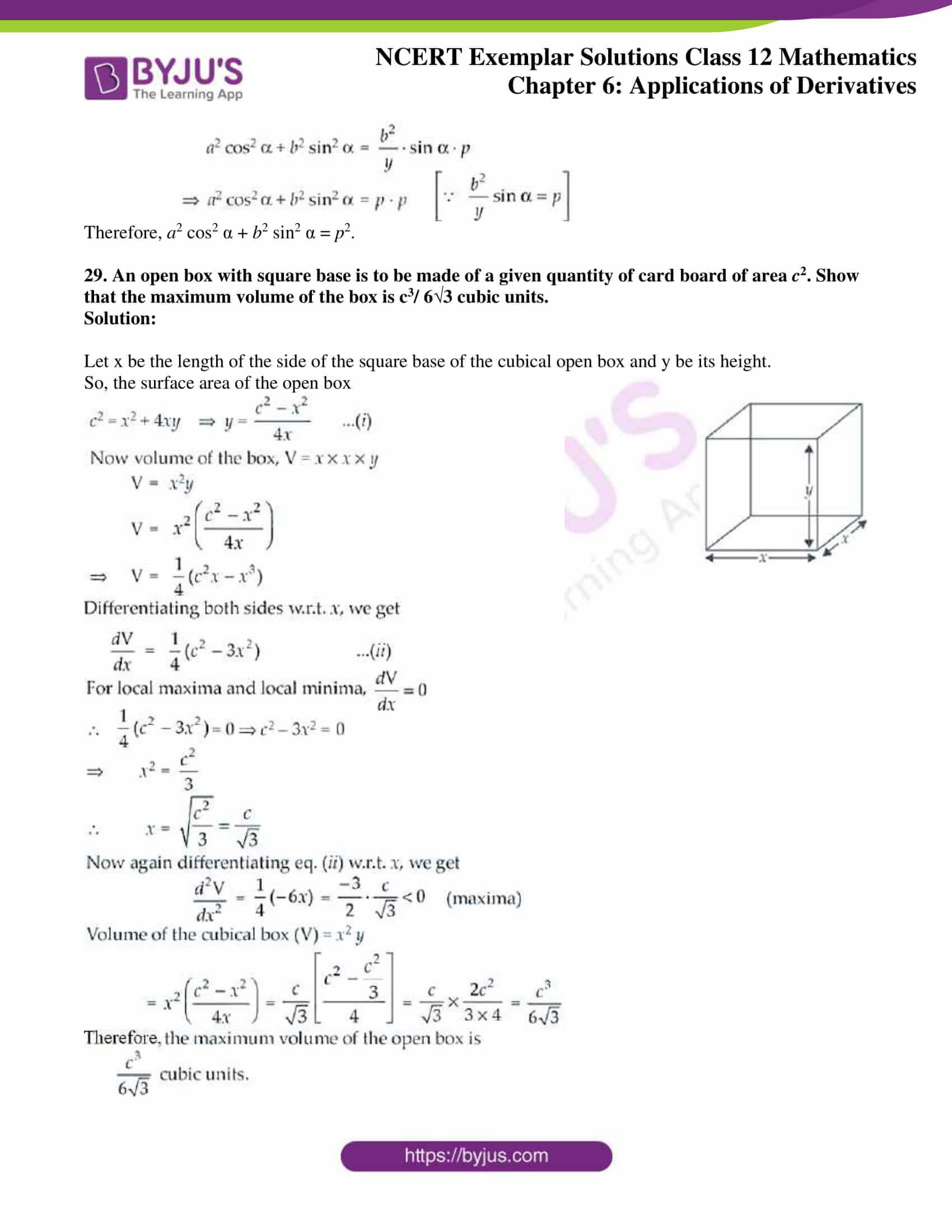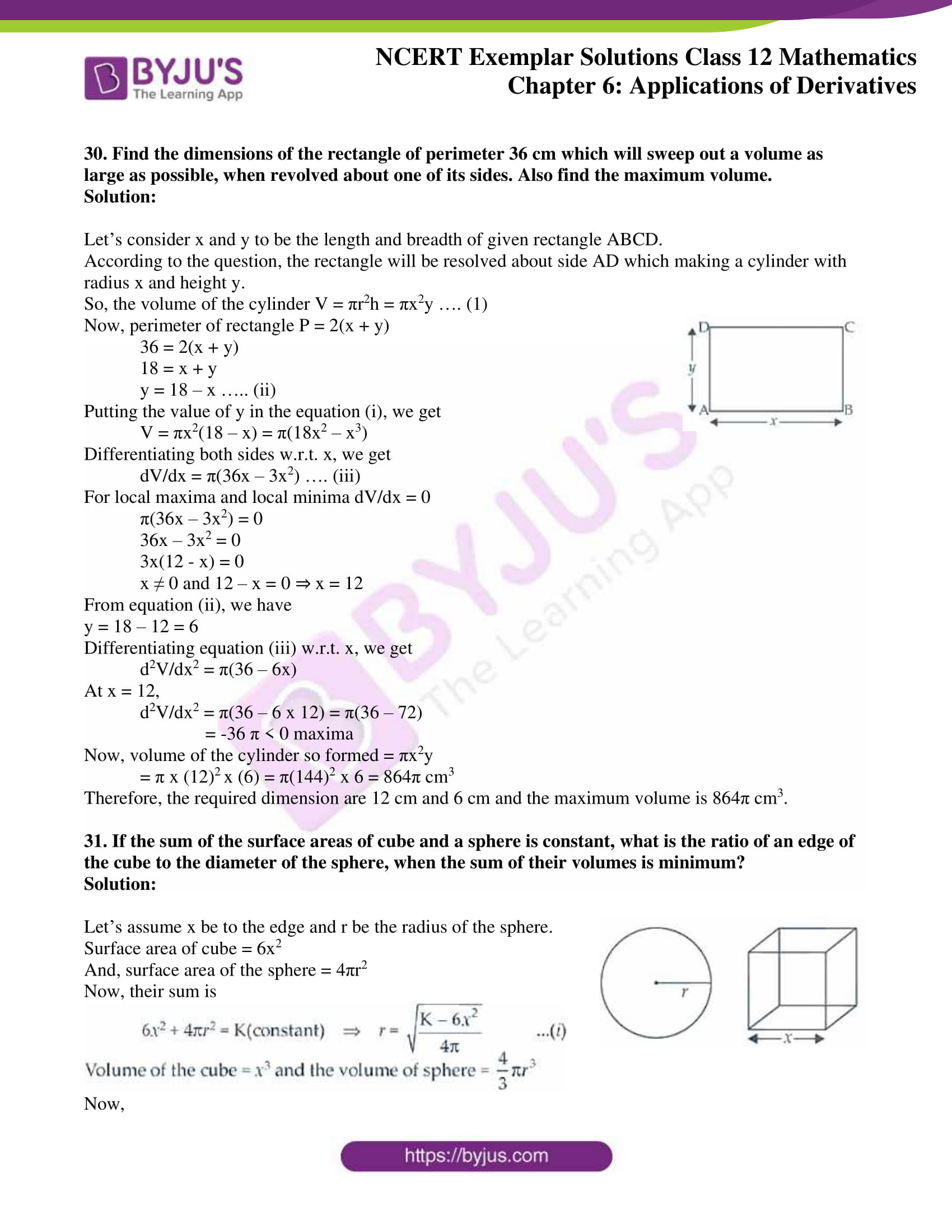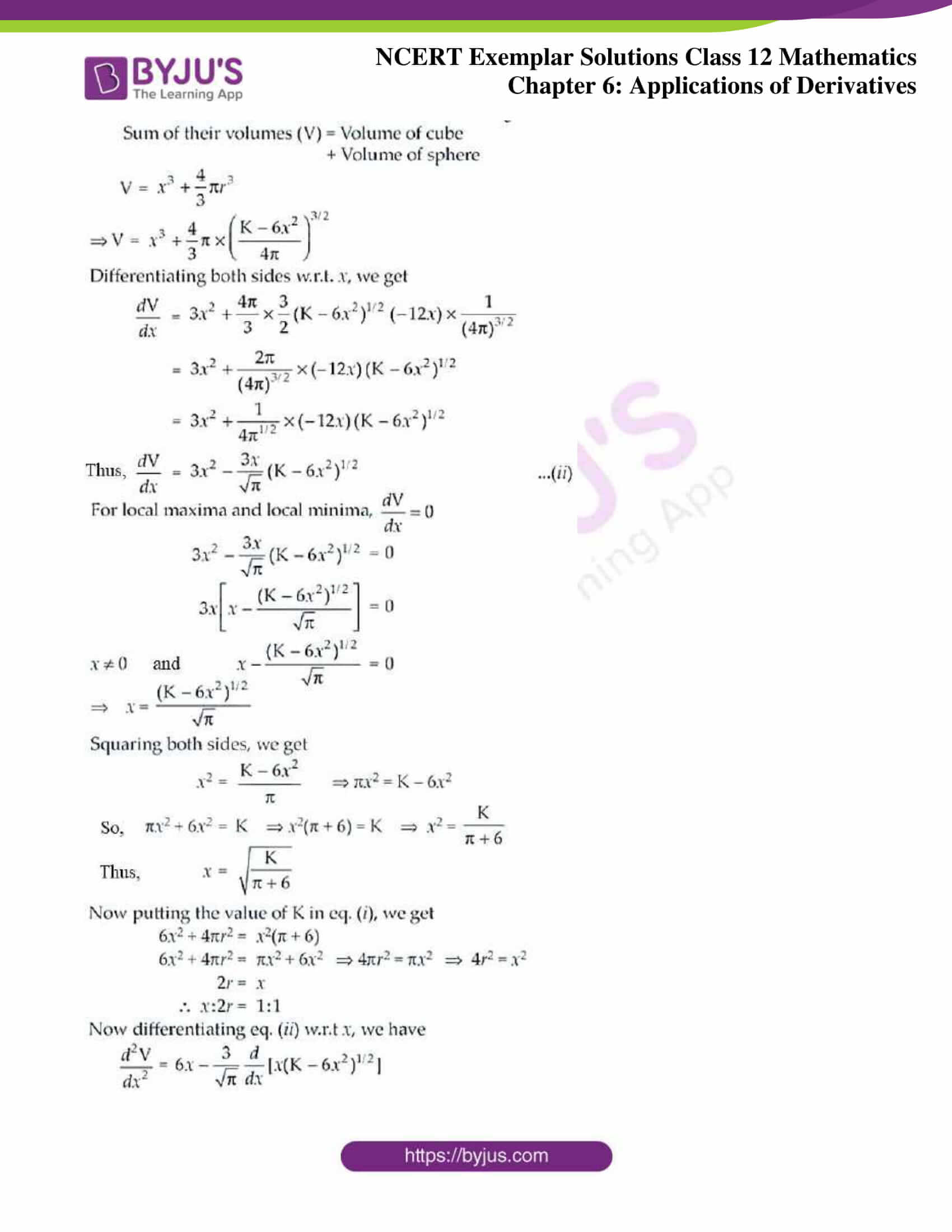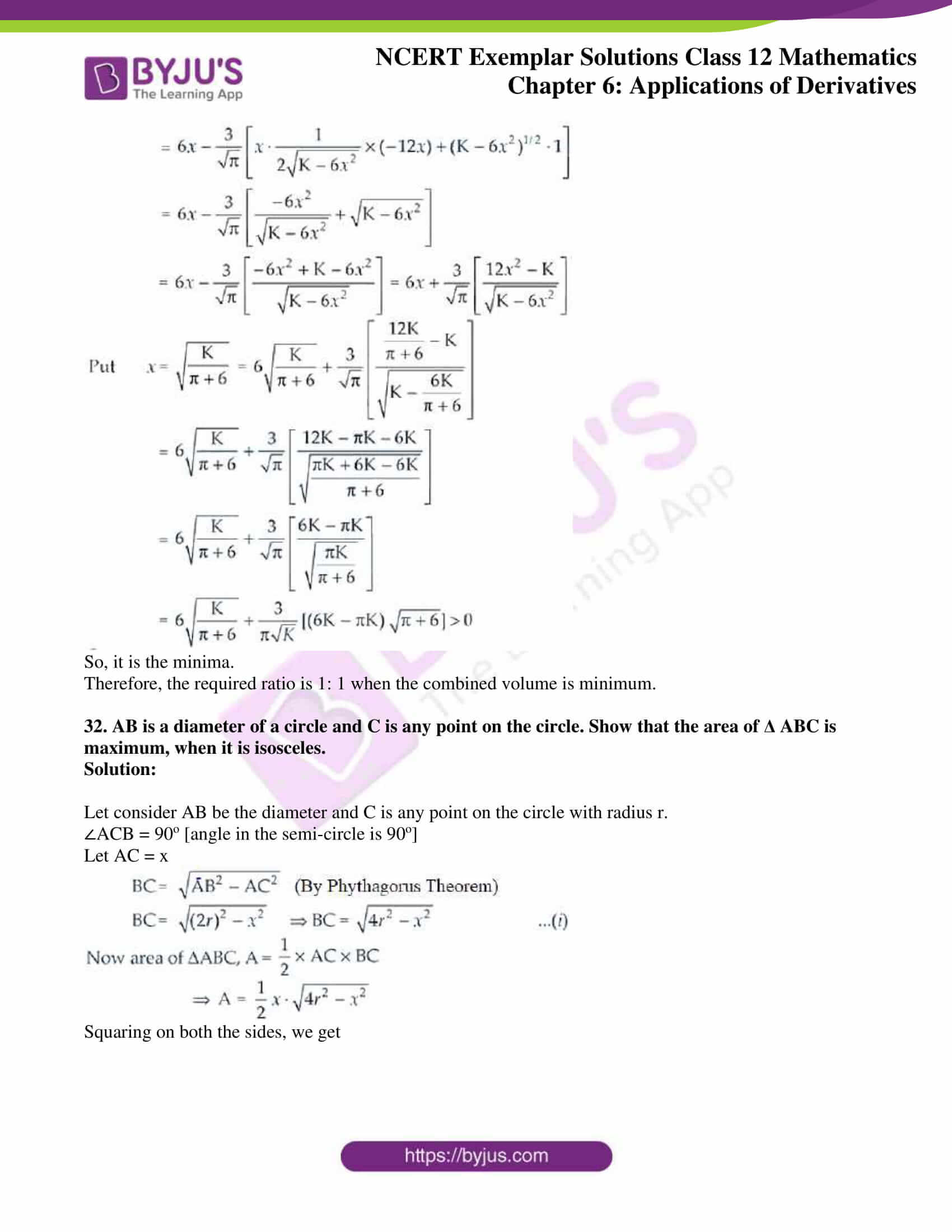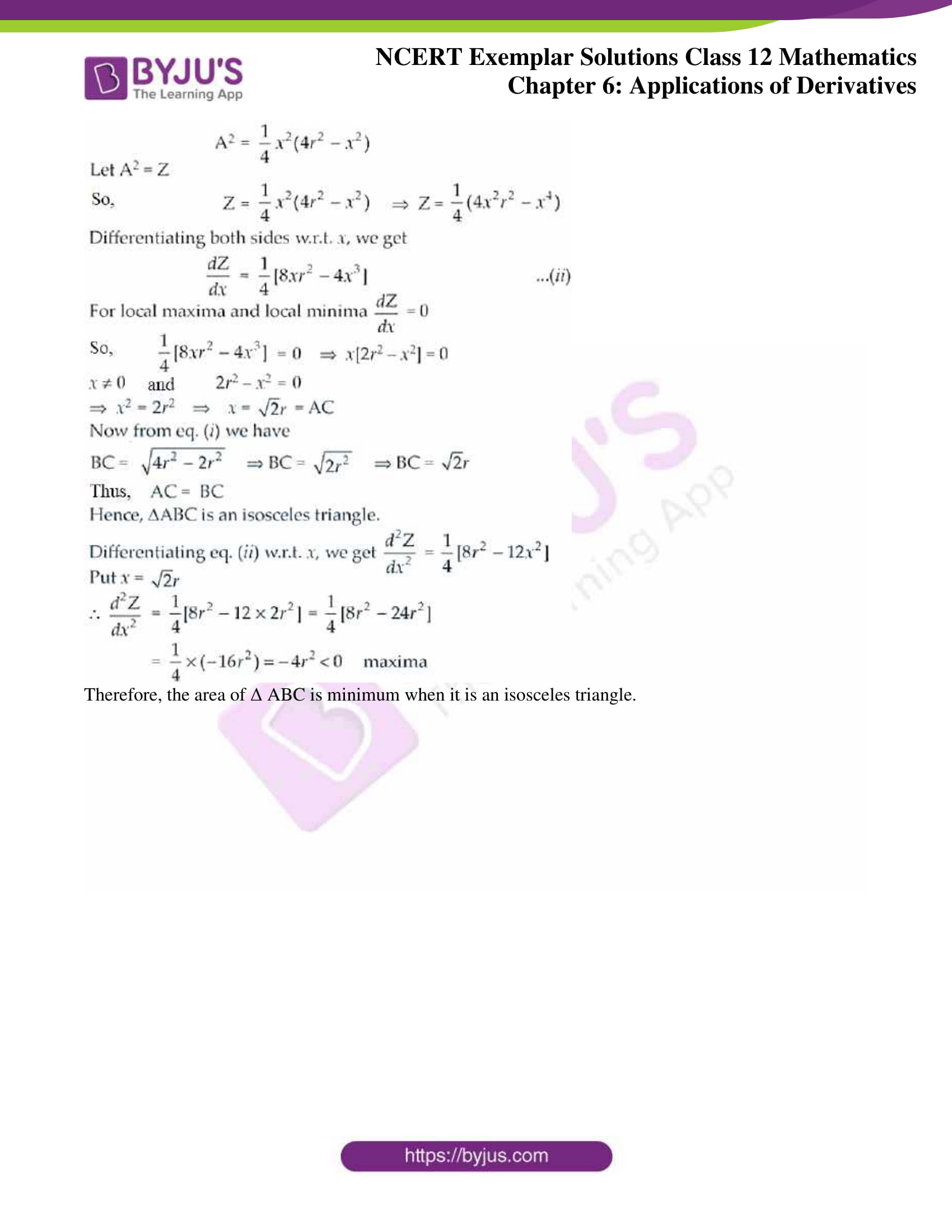### Access Answers to the NCERT Exemplar Class 12 Maths Chapter 6 Application of Derivatives

Exercise 6.3 Page No: 135

1. A spherical ball of salt is dissolving in water in such a manner that the rate of decrease of the volume at any instant is proportional to the surface. Prove that the radius is decreasing at a constant rate.

Solution:

Given, a spherical ball of salt

Then, the volume of ball V = 4/3 πr3 where r = radius of the ball

Now, according to the question we have

dV/dt ∝ S, where S = surface area of the ball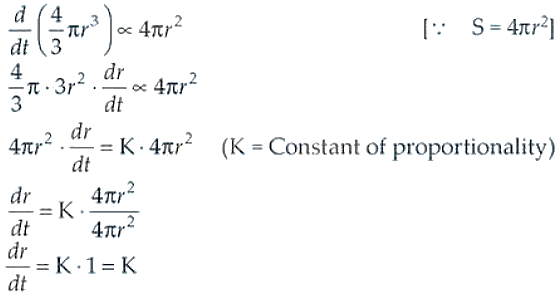Therefore, the radius of the ball is decreasing at constant rate.

2. If the area of a circle increases at a uniform rate, then prove that perimeter varies inversely as the radius.

Solution:

We know that the area of circle, A = πr2, where r = radius of the circle

And, perimeter = 2πr

According to the question, we have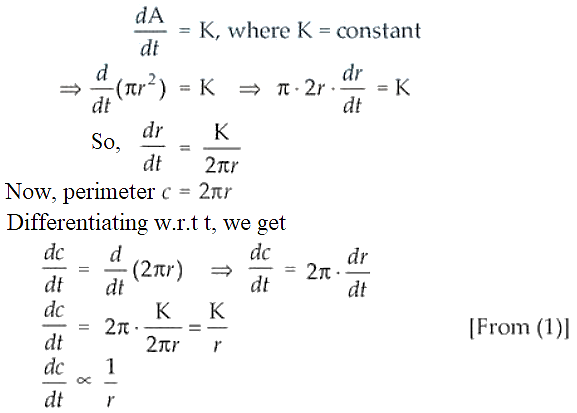Therefore, it’s seen that the perimeter of the circle varies inversely as the radius of the circle.

3. A kite is moving horizontally at a height of 151.5 meters. If the speed of kite is

10 m/s, how fast is the string being let out; when the kite is 250 m away from

the boy who is flying the kite? The height of boy is 1.5 m.

Solution:

Given,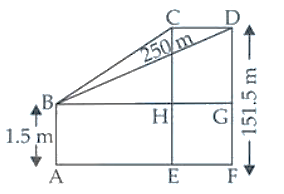Height of the kite(h) = 151.5 m

Speed of the kite(V) = 10 m/s

Let FD be the height of the kite and AB be the height of the kite and AB be the height of the boy.

Now, let AF = x m

So, BG = AF = x

And, dx/dt = 10 m/s

From the figure, it’s seen that

GD = DF – GF = DF – AB

= (151.5 – 1.5) m = 150 m [As AB = GF]

Now, in ∆ BDG

BG2 + GD2 = BD2 (By Pythagoras Theorem)

x2 + (150)2 = (250)2

x2 + 22500 = 62500

x2 = 62500 – 22500 = 40000

x = 200 m

Let initially the length of the string be y m

So, in ∆ BDG

BG2 + GD2 = BD2

x2 + (150)2 = y2

Differentiating both sides w.r.t., t, we have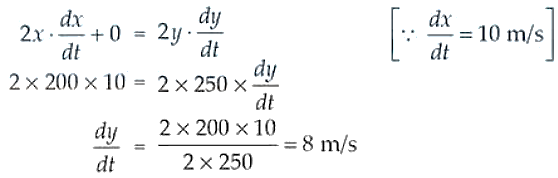Therefore, the rate of change of the length of the string is 8 m/s.

4. Two men A and B start with velocities v at the same time from the junction of

two roads inclined at 45° to each other. If they travel by different roads, find

the rate at which they are being separated.

Solution:

Let’s consider P to be any point at which the two roads are inclined at an angle of 45o.

Now, two men A and B are moving along the roads PA and PB respectively with same speed ‘V’.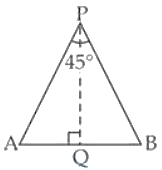And, let A and B be their final positions such that AB = y

∠APB = 45o and they move with the same speed.

So, ∆APB is an isosceles triangle.

Now, draw PQ ⊥ AB.

We have, AB = y

So, AQ = y/2 and PA = PA = x (assumption)

And, ∠APQ = ∠BPQ = 45o/2 = 22.5o

[As the altitude drawn from the vertex of an isosceles ∆, bisects the base]

Now, in right ∆APQ

sin 22.5o = AQ/AP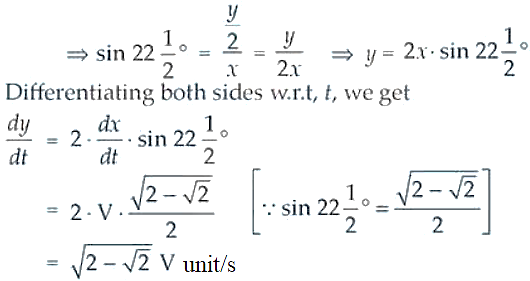Therefore, the rate of their separation is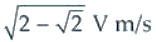5. Find an angle q, 0 < q < /2, which increases twice as fast as its sine.

Solution:

According to the question, we have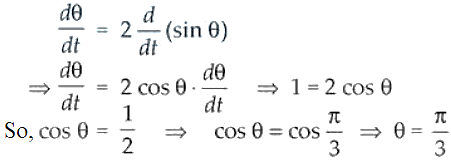Therefore, the required angle is π/3.

6. Find the approximate value of (1.999)5.

Solution:

(1.999)5 = (2 – 0.001)5

Let x = 2 and ∆x = -0.001

Also, let y = x5

Differentiating both sides w.r.t, x, we get

dy/dx = 5x4 = 5(2)4 = 80

Now, ∆y = (dy/dx). ∆x = 80. (-0.001) = -0.080

And, (1.999)5 = y + ∆y

= x5 – 0.080 = (2)5 – 0.080 = 32 – 0.080 = 31.92

Therefore, approximate value of (1.999)5 is 31.92

7. Find the approximate volume of metal in a hollow spherical shell whose internal and external radii are 3 cm and 3.0005 cm, respectively.

Solution:

Given,

The internal radius r = 3 cm

And, external radius R = r + ∆r =3.0005 cm

∆r = 3.0005 – 3 = 0.0005 cm

Let y = r3 ⇒ y + ∆y = (r + ∆r)3 = R3 = (3.0005)3

Differentiating both sides w.r.t., r, we get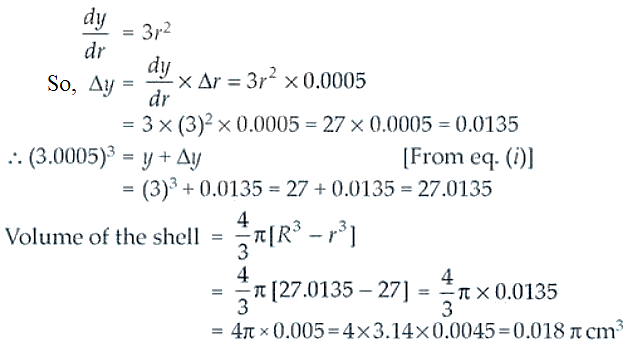Therefore, the approximate volume of the metal in the shell is 0.018π cm3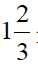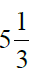8. A man, 2m tall, walks at the rate of m/s towards a street light which is m above

the ground. At what rate is the tip of his shadow moving? At what rate is the length of the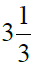shadow changing when he is m from the base of the light?

Solution:

Let AB is the height of street light post and CD is the height of the man such that

AB = 5(1/3) = 16/3 m and CD = 2 m

Let BC = x length (the distance of the man from the lamp post)

And CE = y is the length of the shadow of the man at any instant.

It’s seen from the figure that,

∆ ABE ~ ∆ DCE [by AAA similarity criterion]

Now, taking ratio of their corresponding sides, we have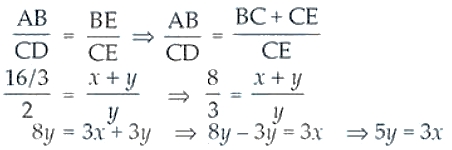Differentiating both sides w.r.t, t, we have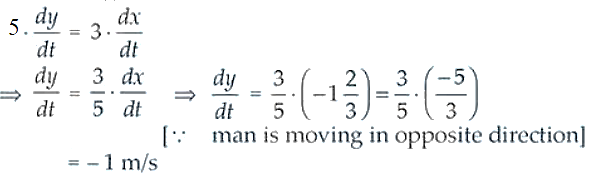So, the length of shadow is decreasing at the rate of 1 m/s.

Now, let u = x + y

(where, u = distance of the tip of shadow from the light post)

On differentiating both sides w.r.t. t, we get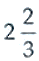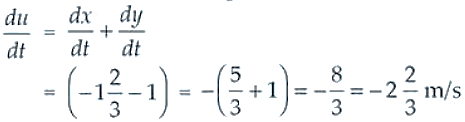Therefore, the tip of the shadow is moving at the rate of m/s towards the light post and the length of shadow decreasing at the rate of 1 m/s.

9. A swimming pool is to be drained for cleaning. If L represents the number of litres of water in the pool t seconds after the pool has been plugged off to drain and L = 200 (10 – t)2. How fast is the water running out at the end of 5 seconds? What is the average rate at which the water flows out during the first 5 seconds?

Solution:

Given, L = 200(10 – t)2 where L represents the number of liters of water in the pool.

On differentiating both the sides w.r.t, t, we get

dL/dt = 200 x 2(10 – t) (-1) = -400(10 – t)

But, the rate at which the water is running out

= -dL/dt = 400(10 – t)

Now, rate at which the water is running after 5 seconds will be

= 400 x (10 – 5) = 2000 L/s (final rate)

T = 0 for initial rate

= 400 (10 – 0) = 4000 L/s

So, the average rate at which the water is running out is given by

= (Initial rate + Final rate)/ 2 = (4000 + 2000)/ 2 = 6000/2 = 3000 L/s

Therefore, the required rate = 3000 L/s

10. The volume of a cube increases at a constant rate. Prove that the increase in its surface area varies inversely as the length of the side.

Solution:

Let’s assume x to be the length of the cube.

So, the volume of the cube V = x3 …. (1)

Given that, dV/dt = K

Now, on differentiating the equation (1) w.r.t. t, we get

dV/dt = 3x2. dx/dt = K (constant)

So, dx/dt = K/3x2

Now,

Surface area of the cube, S = 6x2

Differentiating both sides w.r.t. t, we get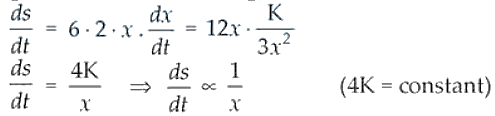Therefore, the surface area of the cube varies inversely as the length of the side.

11. x and y are the sides of two squares such that y = x x2. Find the rate of change of the area of second square with respect to the area of first square.

Solution:

Let’s consider the area of the first square A1 = x2

And, area of the second square be A2 = y2

Now, A1 = x2 and A2 = y2 = (x – x2)2

Differentiating both A1 and A2 w.r.t. t, we get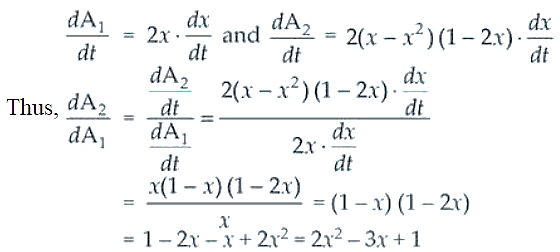Therefore, the rate of change of area of the second square with respect to first is 2x2 – 3x + 1.

12. Find the condition that the curves 2x = y2 and 2xy = k intersect orthogonally.

Solution:

It’s seen that the given curves are equation of two circles.

2x = y2 ….. (1) and

2xy = k ….. (2)

We know that, two circles intersect orthogonally if the angle between the tangents drawn to the two circles at the point of their intersection is 90o.

Now, differentiating equations (1) and (2) w.r.t. t, we get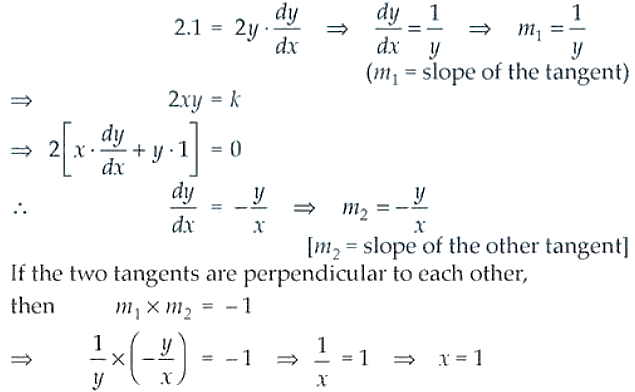Now, solving equations (1) and (2), we have

y = k/2x [From (2)]

Putting the value of y in equation (1),

2x = (k/2x)2 ⇒ 2x = k2/4x2

8x3 = k2

8(1)3 = k2

k2 = 8

Therefore, the required condition is k2 = 8.

13. Prove that the curves xy = 4 and x2 + y2 = 8 touch each other.

Solution:

Given curves are equations of two circles,

xy = 4 ….. (i) and

x2 + y2 = 8 …. (ii)

Different equation (i) w.r.t., x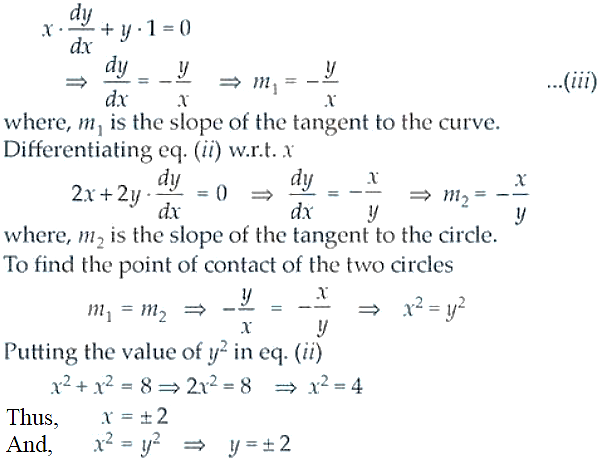Therefore, the point of contact of the two circles are (2, 2) and (-2, 2).

14. Find the co-ordinates of the point on the curve √x + √y = 4 at which tangent is equally inclined to the axes.

Solution:

Equation of the curve is given by, √x + √y = 4

Now, let (x1, y1) be he required point on the curve

So, √x1 + √y1 = 4

On differentiating on both the sides w.r.t. x1, we get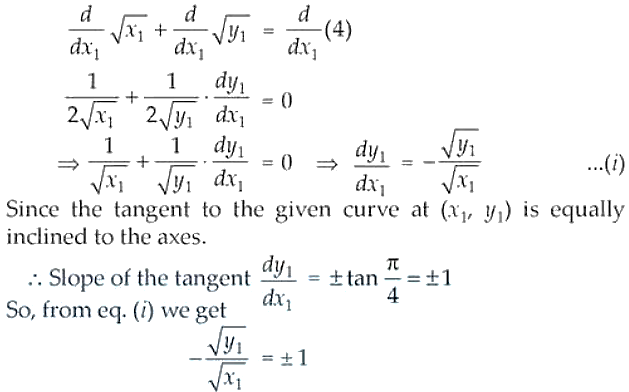On squaring on both the sides, we get

y1/x1 = 1 ⇒ y1 = x1

Now, putting the value of y1 in the given equation of the curve.

x1 + √y1 = 4

x1 + √x1 = 4 ⇒ 2√x1 = 4 ⇒ √x1 = 2 ⇒ x1 = 4

As y1 = x1

⇒ y1 = 4

Therefore, the required point is (4, 4).

15. Find the angle of intersection of the curves y = 4 – x2 and y = x2.

Solution:

The given curves are y = 4 – x2 …. (i) and y = x2 …… (ii)

And, we know that the angle of intersection of two curves is equal to the angle between the tangents drawn to the curves at their point of intersection.

Now, differentiating equations (i) and (ii) w.r.t x, we have

dy/dx = -2x ⇒ m1 = -2x

m1 is the slope of the tangent to the curve (i).

and dy/dx = 2x ⇒ m2 = 2x

m2 is the slope of the tangent to the curve (ii).

So, m1 = -2x and m2 = 2x

On solving equation (i) and (ii), we get

4 – x2 = x2 ⇒ 2x2 = 4 ⇒ x2 = 2 ⇒ x = ±√2

So, m1 = -2x = -2√2 and m2 = 2x = 2√2

Let θ be the angle of intersection of two curves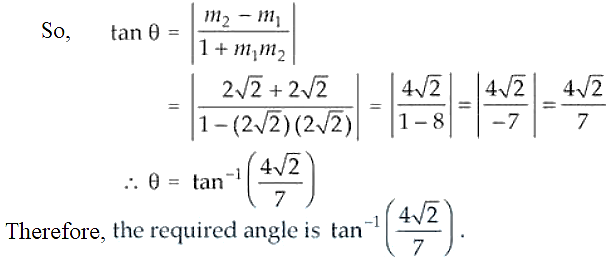16. Prove that the curves y2 = 4x and x2 + y2 – 6x + 1 = 0 touch each other at the point (1, 2).

Solution:

Given curve equations are: y2 = 4x …. (1) and x2 + y2 – 6x + 1 = 0 ….. (2)

Now, differentiating (i) w.r.t. x, we get

2y.(dy/dx) = 4 ⇒ dy/dx = 2/y

Slope of tangent at (1, 2), m1 = 2/2 = 1

Differentiating (ii) w.r.t. x, we get

2x + 2y.(dy/dx) – 6 = 0

2y. dy/dx = 6 – 2x ⇒ dy/dx = (6 – 2x)/ 2y

Hence, the slope of the tangent at the same point (1, 2)

⇒ m2 = (6 – 2 x 1)/ (2 x 2) = 4/4 = 1

It’s seen that m1 = m2 = 1 at the point (1, 2).

Therefore, the given circles touch each other at the same point (1, 2).

17. Find the equation of the normal lines to the curve 3x2y2 = 8 which are parallel to the line x + 3y = 4.

Solution:

Given curve, 3x2y2 = 8

Differentiating both sides w.r.t. x, we get

6x – 2y. dy/dx = 0 ⇒ -2y(dy/dx) = -6x ⇒ dy/dx = 3x/y

So, slope of the tangent to the given curve = 3x/y

Thus, the normal to the curve = -1/(3x/y) = -y/3x

Now, differentiating both sides of the given line x + 3y = 4, we have

1 + 3.(dy/dx) = 0

dy/dx = -1/3

As the normal to the curve is parallel to the given line x + 3y = 4

We have, -y/3x = -1/3 ⇒ y = x

On putting the value of y in 3x2 – y2 = 8, we get

3x2 – x2 = 8

2x2 = 8 ⇒ x2 = 4 ⇒ x = ±2

So, y = ±2

Thus, the points on the curve are (2, 2) and (-2, -2).

Now, the equation of the normal to the curve at (2, 2) is given by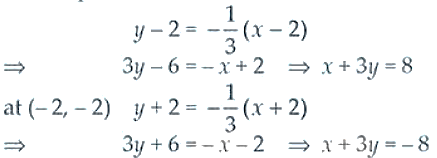Therefore, the required equations are x + 3y = 8 and x + 3y = -8.

18. At what points on the curve x2 + y2 – 2x – 4y + 1 = 0, the tangents are parallel to the y-axis?

Solution:

Given, the equation of the curve is x2 + y2 – 2x – 4y + 1 = 0 ….. (i)

Differentiating both the sides w.r.t. x, we get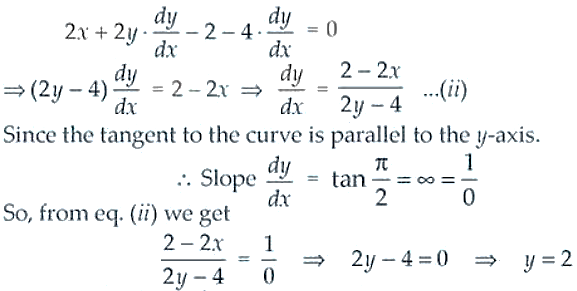Now putting the value of y in equation (i), we get

x2 + (2)2 – 2x – 8 + 1 = 0

x2 – 2x + 4 – 8 + 1 = 0

x2 – 2x – 3 = 0 ⇒ x2 – 3x + x – 3 = 0

x(x – 3) + 1(x – 3) = 0 ⇒ (x – 3)(x + 1) = 0

x = -1 or 3

Therefore, the required points are (-1, 2) and (3, 2).

19. Show that the line x/a + y/b = 1, touches the curve y = b . e-x/a at the point where

the curve intersects the axis of y.

Solution:

Given curve equation, y = b . e-x/a and line equation x/a + y/b = 1

Now, let the coordinates of the point where the curve intersects the y-axis be (0, y1)

Now differentiating y = b . e-x/a both sides w.r.t. x, we get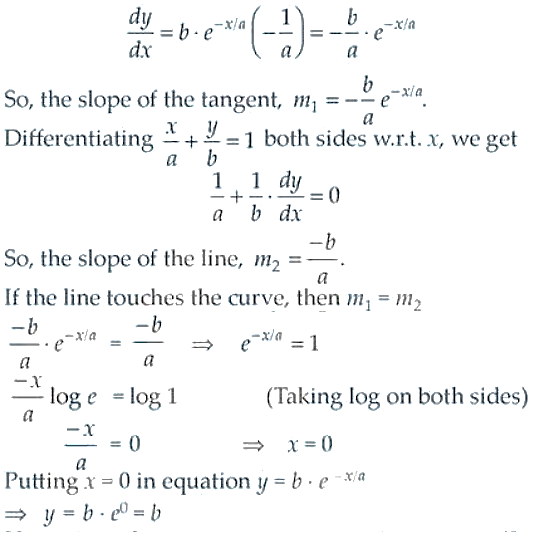Therefore, the equation of the curve intersects at (0, b) which is on the y-axis.

20. Show that f (x) = 2x + cot-1 x + log [√(1 + x2) – x] is increasing in R.

Solution:

Given,

f (x) = 2x + cot-1 x + log [√(1 + x2) – x]

Differentiating both sides w.r.t. x, we get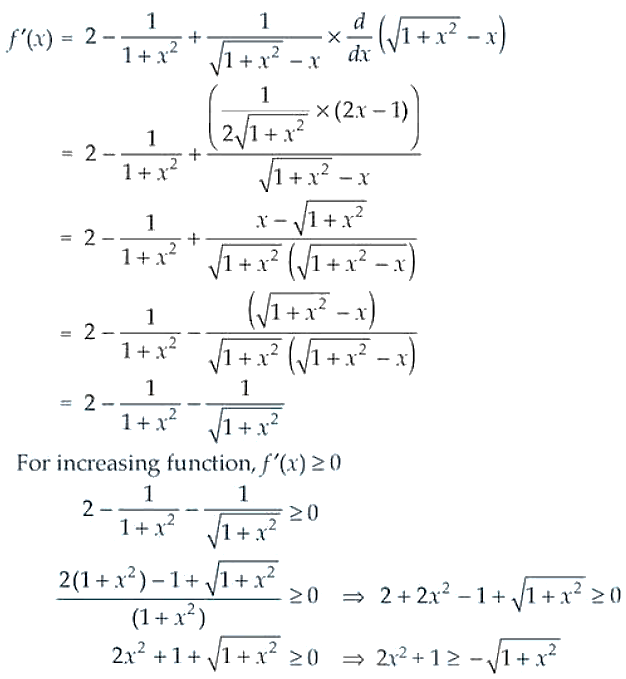On squaring both the sides, we get

4x4 + 1 + 4x2 ≥ 1 + x2

4x4 + 4x2 – x2 ≥ 0

4x4 + 3x2 ≥ 0

x2(4x2 + 3) ≥ 0

The above is true for any value of x ∈ R.

Therefore, the given function is an increasing function over R.

21. Show that for a ³ 1, f (x) = √3 sin x – cos x – 2ax + b is decreasing in R.

Solution:

Given,

f (x) = √3 sin x – cos x – 2ax + b, a ³ 1

On differentiating both sides w.r.t. x, we get

f’ (x) = √3 cos x + sin x – 2a

For increasing function, f’ (x) < 0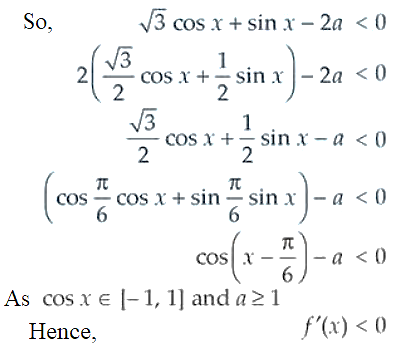Therefore, the given function is decreasing in R.

22. Show that f(x) = tan–1(sin x + cos x) is an increasing function in (0, π/4).

Solution:

Given, f(x) = tan–1(sin x + cos x) in (0, π/4).

Differentiating both sides w.r.t. x, we got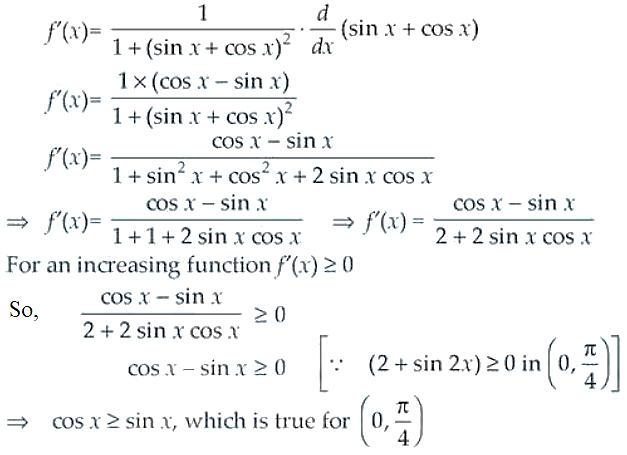Therefore, the given function f(x) is an increasing function in (0, π/4).

23. At what point, the slope of the curve y = – x3 + 3x2 + 9x – 27 is maximum? Also find the maximum slope.

Solution:

Given, curve y = – x3 + 3x2 + 9x – 27

Differentiating both sides w.r.t. x, we get

dy/dx = -3x2 + 6x + 9

Let slope of the curve dy/dx = z

So, z = -3x2 + 6x + 9

Differentiating both sides w.r.t. x, we get

dz/dx = -6x + 6

For local maxima and local minima,

dz/dx = 0

-6x + 6 = 0 ⇒ x = 1

d2z/dx2 = -6 < 0 Maxima

Putting x = 1 in equation of the curve y = (-1)3 + 3(1)2 + 9(1) – 27

= -1 + 3 + 9 – 27 = -16

Maximum slope = -3(1)2 + 6(1) + 9 = 12

Therefore, (1, -16) is the point at which the slope of the given curve is maximum and maximum slope = 12.

24. Prove that f (x) = sin x + √3 cos x has maximum value at x = π/6.

Solution: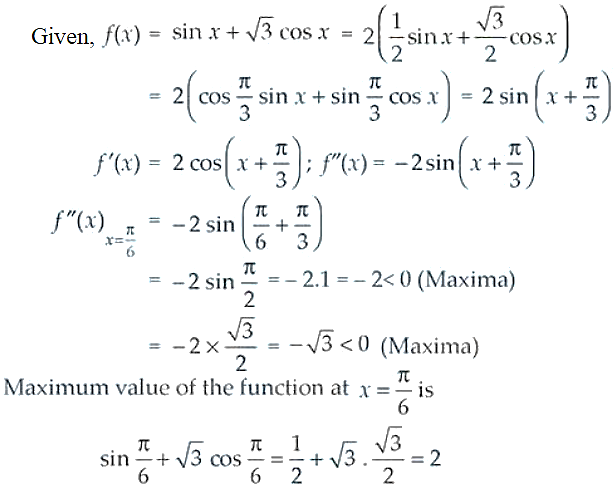Therefore, the given function has maximum value at x = π/6 and the maximum value is 2.

25. If the sum of the lengths of the hypotenuse and a side of a right angled triangle is given, show that the area of the triangle is maximum when the angle between them is π/3.

Solution: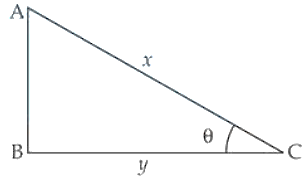Let ∆ABC be the right-angled triangle in which ∠B = 90o

Let AC = x, BC = y

So, AB = √(x2 + y2)

∠ACB = θ

Let z = x + y (given)

Now, the area of ∆ABC = ½ x AB x BC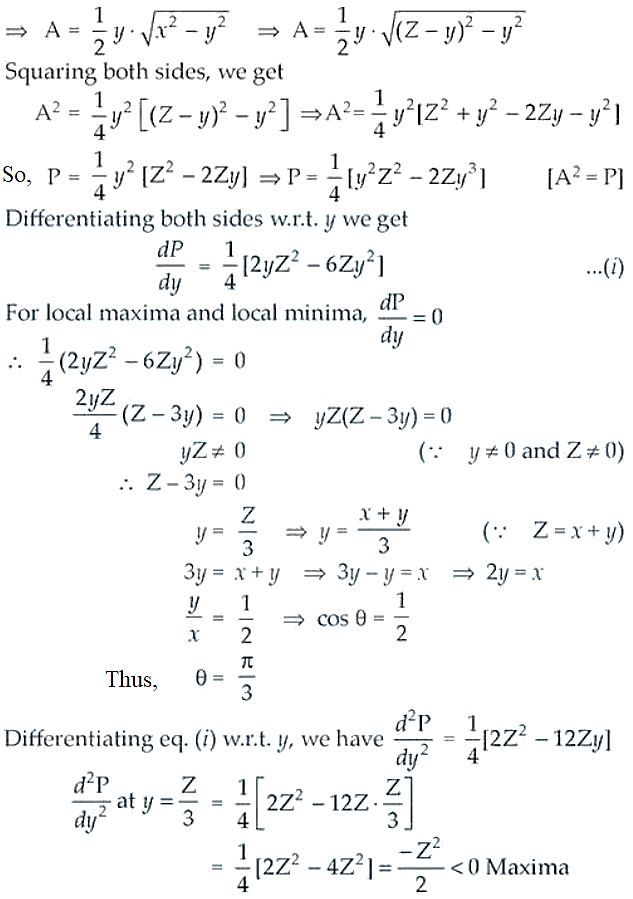Therefore, the area of the given triangle is maximum when the angle between its hypotenuse and a side is π/3.

26. Find the points of local maxima, local minima and the points of inflection of the function f (x) = x5 – 5x4 + 5x3 – 1. Also find the corresponding local maximum and local minimum values.

Solution:

Given, f (x) = x5 – 5x4 + 5x3 – 1

Differentiating the function,

f’ (x) = 5x4 – 20x3 + 15x2

For local maxima and local minima, f’ (x) = 0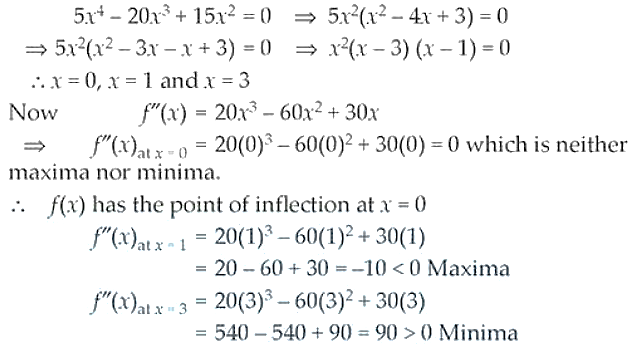The maximum value of the function at x = 1

f(x) = (1)5 – 5(1)4 + 5(1)3 – 1

= 1 – 5 + 5 – 1 = 0

The minimum value at x = 3 is

f(x) = (3)5 – 5(3)4 + 5(3)3 – 1

= 243 – 405 + 135 – 1

= 378 – 406 = -28

Therefore, the function has its maxima at x = 1 and the maximum value = 0 and its has minimum value at x = 3 and its minimum value is -28.

27. A telephone company in a town has 500 subscribers on its list and collects fixed charges of Rs 300/- per subscriber per year. The company proposes to increase the annual subscription and it is believed that for every increase of Rs 1/- one subscriber will discontinue the service. Find what increase will bring maximum profit?

Solution:

Let’s consider that the company increases the annual subscription by Rs x.

So, x is the number of subscribers who discontinue the services.

Total revenue, R(x) = (500 – x) (300 + x)

= 150000 + 500x – 300x – x2

= -x2 + 200x + 150000

Differentiating both sides w.r.t. x, we get R’(x) = -2x + 200

For local maxima and local minima, R’(x) = 0

-2x + 200 = 0 ⇒ x = 100

R’’(x) = -2 < 0 Maxima

So, R(x) is maximum at x = 100

Therefore, in order to get maximum profit, the company should increase its annual subscription by Rs 100.

28. If the straight-line x cos α + y sin α = p touches the curve x2/a2 + y2/b2 = 1, then prove that a2 cos2 α + b2 sin2 α = p2.

Solution:

The given curve is x2/a2 + y2/b2 = 1 and the straight-line x cos α + y sin α = p

Differentiating equation (i) w.r.t. x, we get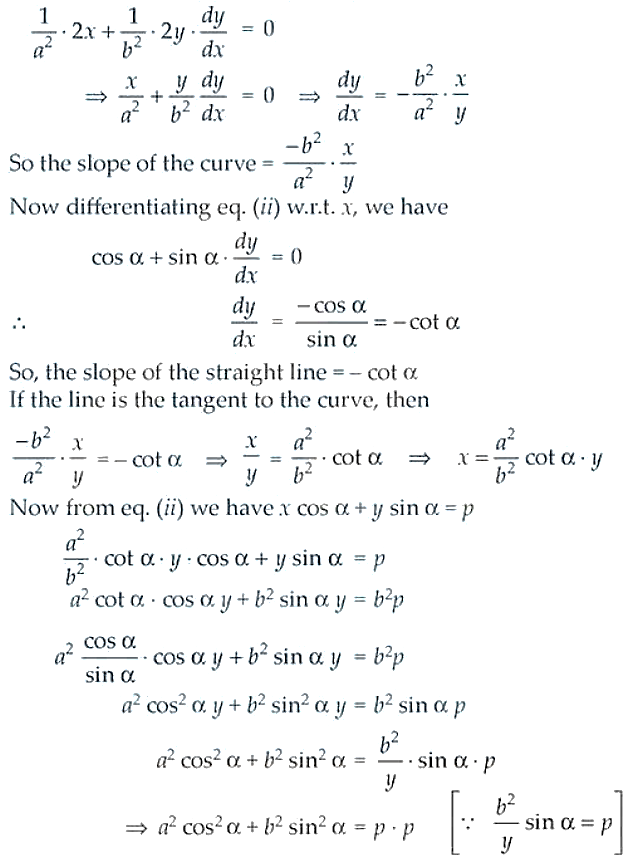Therefore, a2 cos2 α + b2 sin2 α = p2.

29. An open box with square base is to be made of a given quantity of card board of area c2. Show that the maximum volume of the box is c3/ 6√3 cubic units.

Solution:

Let x be the length of the side of the square base of the cubical open box and y be its height.

So, the surface area of the open box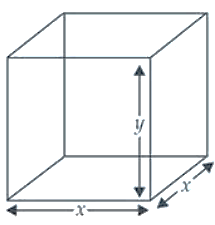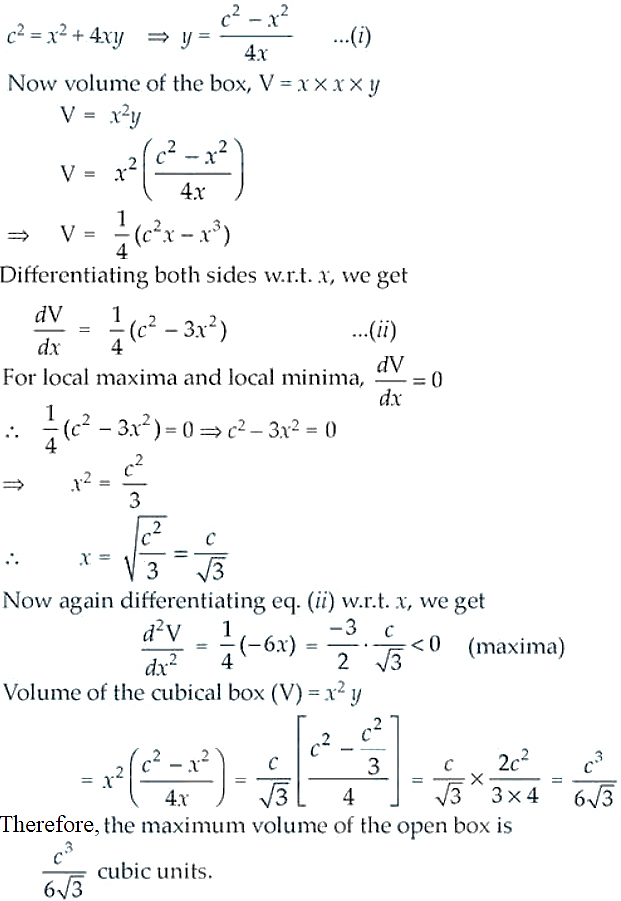30. Find the dimensions of the rectangle of perimeter 36 cm which will sweep out a volume as large as possible, when revolved about one of its sides. Also find the maximum volume.

Solution:

Let’s consider x and y to be the length and breadth of given rectangle ABCD.

According to the question, the rectangle will be resolved about side AD which making a cylinder with radius x and height y.

So, the volume of the cylinder V = πr2h = πx2y …. (1)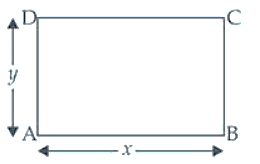Now, perimeter of rectangle P = 2(x + y)

36 = 2(x + y)

18 = x + y

y = 18 – x ….. (ii)

Putting the value of y in the equation (i), we get

V = πx2(18 – x) = π(18x2 – x3)

Differentiating both sides w.r.t. x, we get

dV/dx = π(36x – 3x2) …. (iii)

For local maxima and local minima dV/dx = 0

π(36x – 3x2) = 0

36x – 3x2 = 0

3x(12 – x) = 0

x ≠ 0 and 12 – x = 0 ⇒ x = 12

From equation (ii), we have

y = 18 – 12 = 6

Differentiating equation (iii) w.r.t. x, we get

d2V/dx2 = π(36 – 6x)

At x = 12,

d2V/dx2 = π(36 – 6 x 12) = π(36 – 72)

= -36 π < 0 maxima

Now, volume of the cylinder so formed = πx2y

= π x (12)2 x (6) = π(144)2 x 6 = 864π cm3

Therefore, the required dimension are 12 cm and 6 cm and the maximum volume is 864π cm3.

31. If the sum of the surface areas of cube and a sphere is constant, what is the ratio of an edge of the cube to the diameter of the sphere, when the sum of their volumes is minimum?

Solution: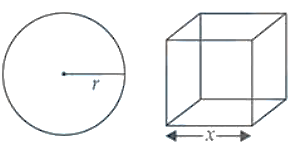Let’s assume x be to the edge and r be the radius of the sphere.

Surface area of cube = 6x2

And, surface area of the sphere = 4πr2

Now, their sum is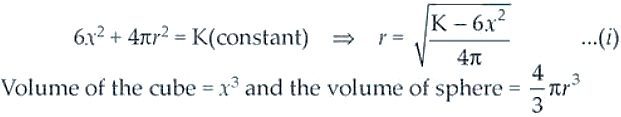Now,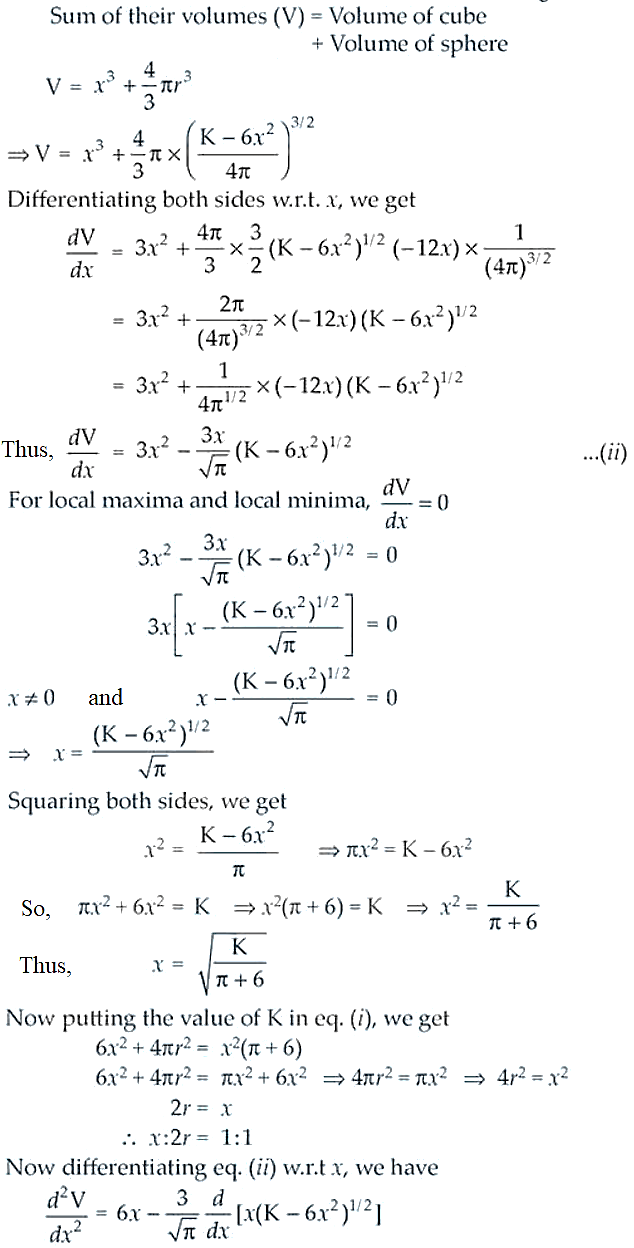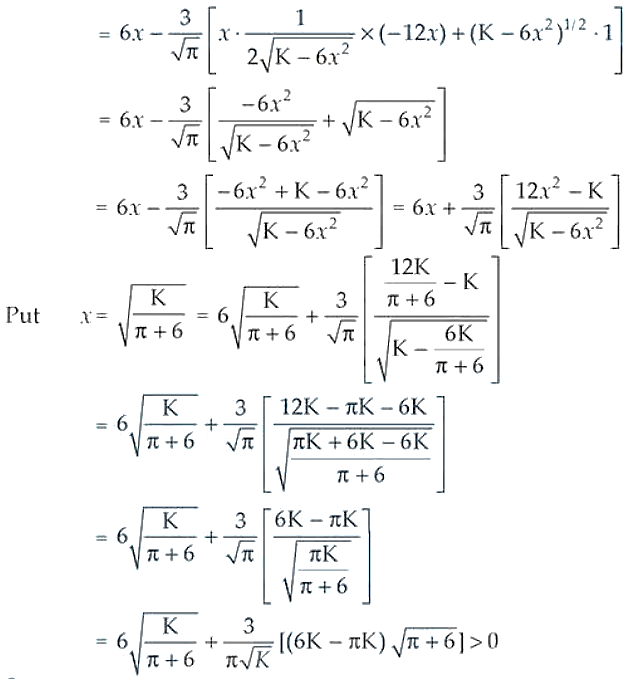So, it is the minima.

Therefore, the required ratio is 1: 1 when the combined volume is minimum.

32. AB is a diameter of a circle and C is any point on the circle. Show that the area of Δ ABC is maximum, when it is isosceles.

Solution:

Let consider AB be the diameter and C is any point on the circle with radius r.

∠ACB = 90o [angle in the semi-circle is 90o]

Let AC = x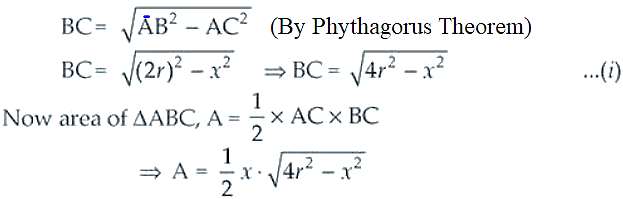Squaring on both the sides, we get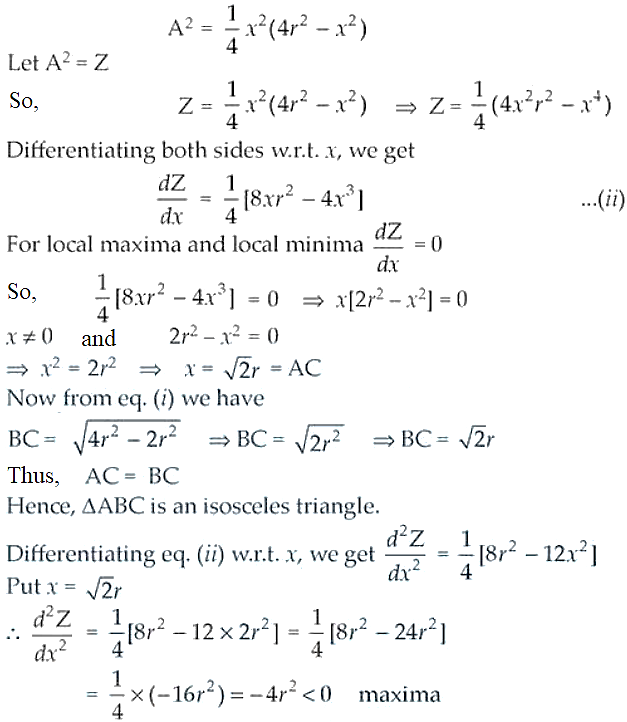Therefore, the area of Δ ABC is minimum when it is an isosceles triangle.